NEU Theory

## NEU Theory

The Nature of Physical Reality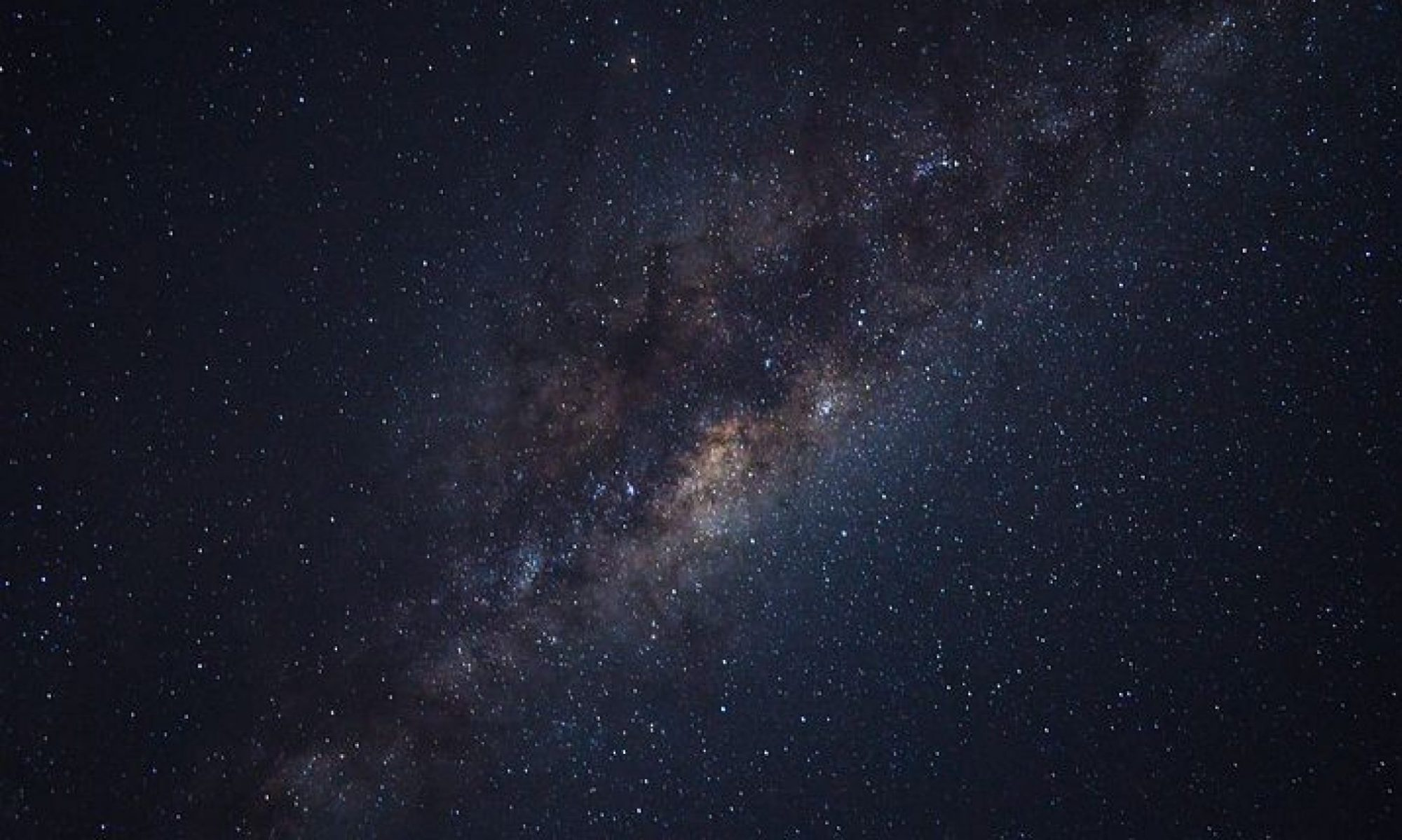### What is the physical foundation of nature and how does it work?

Welcome to Neu Theory – a simple topological model of the universe where perpetual interactions between seven elementary particles, four natural accelerations, and one large number provide a dynamic stable physical foundation to our common existence.

Neu Theory is a model of atoms, space and light, the ordinary stuff of physical reality. Neu Theory is a world model where nature is seen as ultimately simple and dependable, not complicated and indeterminate.

Neu Theory is a world model without singularities, a big bang, spacetime, neutrinos, antimatter, dark matter, dark energy, quarks, and the strong nuclear force. None are needed or provided. It can be shown how our amazing universe can work just fine without them.

The model is grounded in well documented “facts of experience,” that are readily available from multiple sources, for example, physics and chemistry handbooks and science websites. None of the starting empirical data used in this work has been created by the writer. There is no need, as the facts themselves are indisputable. It is the interpretation of these facts that is always the issue.

Questions about nature have been asked by human beings since the beginning of mental coherence. The answers that are currently provided by science, even if they mathematically work to a remarkable precision, are not always consistent or complete. Neu Theory uses the same stubborn irrefutable physical facts as everyone else, however, based on the same empirical data, provides a very different interpretation of all fundamental physical phenomena in one simple interconnected model. The entire theory is on this page. The model is open to be tested and falsified

Neu Theory is a creation of this writer’s imagination. You will not find anything like this anywhere else. The ideas were born thinking about the enigma of gravitation. It started with the question, is there an answer for “falling bodies” that did not require “spacetime”, a cosmic space that can compress, bend and warp, and a flow of time that slows with velocity? Neu Theory provided another simpler answer.

There was also the long standing (1911) mystery of the “missing energy” in beta decay. The objective became to find a solution that did not require using the neutrino particle, first proposed by the physicist Wolfgang Pauli in 1930. The existence of the three “ghost” oscillating neutrinos is critical for current science, because without them, theories on particle physics, such as the “Standard Model”, cannot be sustained.

The term “beta decay”, called the “little bang” in this model, is the spontaneous transformation of the neutron into the proton and electron with a constant reduction of mass. This invariant relationship between the three masses is the empirical foundation and cornerstone of Neu Theory.

In this model the “missing energy” during beta decay, does not become three “fundamental” neutrino particles, whose physical existence can only be indirectly inferred, by observing subatomic events in highly controlled environments. After over sixty years and billions of dollars spent in scientific experimental effort, not one neutrino (despite being considered a fermion, and existing in the zillions) has ever been “caught”, meaning, that a neutrino has never been directly unambiguously observed with some, any, physical property measured, e.g., mass, momentum, spin, etc. In this model it is hypothesized that no neutrino, of any type or flavor, will ever be caught, because the neutrino as a discrete physical particle does not exist in nature. The “missing energy” is real but neutrinos are not.

In Neu Theory the “missing energy” during beta decay, is the birth of ordinary space in the form of an elementary “zomon” particle, a quantum burst of isotropic free rise energy which expands and diffuses-in-place at the accelerating speed of light into universal space, the model calls the Zome. A small explosion that adds a fresh drop of boson space energy to the existing ocean of dynamic cosmic space energy, that is simultaneously expanding and diffusing-in-place at the accelerating speed of light, in all directions throughout the universe.

It is hypothesized, that in principle the zomon can be observed, but only during the brief moment its zome rise energy density is greater than the background rise energy density of space. Neu Theory calculates, that the average little bang zomon detectable volume is within a sphere two meters in diameter. The detectable lifetime is around three billionths of one second.

In this model the elementary photon particles do not move, meaning they are not self-propelled, instead, they are being carried by a perpetually moving space at the accelerating speed of light, in the unique direction of their birth.

The fermion neutrino particle of current science, is the boson zomon particle of dynamic Neu Theory space. All the “missing” energy of beta decay can be accounted for.

The ideas in this work are a contribution to human thought. The writer does not intend to defend or promote Neu Theory. The model is being presented as is. If there is truth here, it will eventually become apparent to others. If not, so be it. Right or wrong, when a different path is traveled, much can still be learned from the journey.

A good way to start is with Simple Object Topology, an intuitive method to easily understand the shape of real objects. Look at the diagram “Three Primal Shapes“. In the Neu Theory model all of nature’s physical objects are built from large number additions of microscopic balls, shells, and donuts. No other shapes are needed.

Curious readers with questions about the smallest and largest things in nature are encouraged to begin with a completely open mind (a tabula rasa) and decide for themselves. While these concepts can be easily understood, they are so different from current scientific thought, it will initially require some effort and a paradigm shift. For a while, forget what you think you know. There is much to relearn with this model. For example, please look at the diagram “Direction of Force – The Cantilevered Pendulums Experiment.

The question is, what will happen, A or B? One way or another, this thought experiment speaks for itself. Neu Theory uses B.

#### Neu Theory is a graphic model, not a mathematical theory

Neu Theory is not a mathematical model. There are no complex equations trying to solve something. Nothing is being proved or disproved. Simple arithmetic, and the ability to visualize three simple shapes, are all that is needed to understand this model.

Neu Theory is a graphic subatomic and cosmological model. All of Nature’s fundamental physical forms can be visualized, quantified, and measured. The model is a simple way of looking at our common underlying physical structure that seems to work.

Only two numbers are required by the model. Each has quantifiable and measurable physical properties. The first, is the number one, identified as the ordinary neutron and called quantum n. The second, is an invariant collection of quantum n’s called N Prime.

Quantum n‘s exist in one of two natural states, the neutral a-state as the neutron, and the electric b-state as hydrogen. An increase in one state requires a decrease in the other. Both states must add up to the cosmic number N Prime.

The two states topologically combine to synthesize the elements and isotopes using stars as factories. The stars themselves are part of a larger atomic matter/energy cycle maintained by all the galaxies in the universe. Everything in nature works and is built around this.

We can only estimate what the cosmic population of neutrons is, but it is fixed and will never change. It is hypothesized that starting at the primal level there is only addition in nature. All fractions of n must add up to one, and all ones must add up to N. The conservation of neu number, is nature’s Certainty Principle.

We shall see how a large number of three dynamic primal forms of matter, simple objects with invariant properties, following simple rules, given enough time, can create physical objects and environments, that allow for the emergence of complex bodies, starting with atoms, and from this comes, you, me, and all the inventive technology that surrounds us.

### The Homeostatic Universe

The universe is modeled as a physical organism made of matter, energy and movement/synergy fields, that maintain a state of physical homeostasis.

The homeostatic universe is imagined as a primal cosmic being, a pure number called N Prime, that exists in a steady state within a stable volume. Eternal existence is powered by a natural internal acceleration, identified as a very small uniform increase called “a” to the absolute speed of light “c“. The natural acceleration a is a perpetual one-way primal force of nature, which generates four physical accelerations, two with matter forms (magnetism and gravity), and two with energy forms (electricity and space). These four physical accelerations become the four g-forces of nature that continuously drive the cosmos.

Perpetual uniform universal acceleration a is the physical identity of the phenomenon we call the arrow of time.

In this model, the universe is not getting bigger, there is no outward expansion into some nameless void. The volume of space, nature’s largest physical object, fluctuates within narrow self regulating limits. The relative distance between far away galaxies is not increasing. The average density of matter is not decreasing. There is no “Hubble Flow” of space. There s no “dark energy” that accelerates this flow.

The physical meaning of space, light and redshift in Neu Theory is entirely different than what is currently accepted.

In this model, cosmic homeostasis is maintained by an one-way atomic matter/energy cycle, using a web of an estimated 500 billion galaxies of stars, each with super-massive electric supercells (black holes) at their centers, operating as giant regional recycling plants.

Please look at the diagrams, “The Milky Way Electric Supercell“, and “Galaxy Electric Supercell Dynamics (Black Hole)“.

Look at the photograph at the top of this page. This looks towards the center of the Milky Way Galaxy (Sagittarius A*), which is estimated to have a 4.1 million solar mass core equal to ~8.16 x 1036 kg. This amount of mass is equal to a neu number of 4.87 x 1063.

In this model, the Milky Way supercluster core is inhabited by a large number of matter cells, called neucleons, that are tightly packed together as a dense ball, similar to a gigantic atomic nucelus. Most of the neucleons in the core ball are hypothesized to be deuteron cells. A smaller number are neutron cells. The actual deuteron/neutron ratio of the milky way supercluster core is yet to be determined.

Using the neu number/neucleon ratio of Iron-56 (56/30), it is estimated that there are ∼2.6 x 1063 neucleon cells in the milky way core, with 87% deuterons and 13% neutrons.

Using a theoretical specific core neucleon packing density at 73.98% of absolute density, similar to the 126 neucleons (82 deuterons + 44 neutrons) of Lead-208, the Milky Way core  has a calculated volume of 1.65 x 1019 m3, equal to a sphere with a diameter of 3,156 kilometers.

Imagine this as you look at the night sky. The Milky Way supercell matter core, the heart of our galaxy, with a mass of 4.1 million suns, is about 9% smaller in diameter than our Moon.

All ∼ 2.27 x 1063 deuteron positive electric charge shells have migrated above the neutral neucleonic core surface into one positive electric super shield of free spin energy closing around the matter ball at the accelerating speed of light. The positive electric super shield is estimated to have a finite shell thickness around 75 kilometers. This is the anode of the supercell. Similar to atomic nuclides the supercell core has a net spin/magnetic axis, which becomes the axis of rotation and matter accretion for the galaxy.

The Schwarzschild radius of 4.1 million solar masses is 12 million kilometers. It is hypothesized that at a distance of 18 million kilometers, 1.5 times the Schwarzschild radius (called the “photon sphere”), approximately 1/8 the distance from the earth to the sun, there is a cloud of electrons, a dense shell of ∼ 2.27 x 1063 negatively charged electrons vibrating near the speed of light, surrounding the electric supercell core. This is the cathode of the supercell.

Similar to an atom with its electrons, the electric supercell is essentially a neutral body with only a small residual charge, depending on the exact numbers of deuterons and electrons.

Using Newtonian dynamics (g=Gm/r2) the milky way supercell matter core has a calculated g-rise of approximately 2.2 x 1014 m/s2. In one second the calculated in-place surface acceleration of the core has reached 1.1 x 1014 meters, which is 367,000 times more than the distance light would travel in one second!?

This may seem impossible, until you realize that the g-rise of matter is acceleration, not motion. To measure acceleration one starts from zero (0). Another way to consider it is that, starting from zero, the g-rise of the core surface will reach the speed of light at 299 792 458 m/s, in some small fraction of one second as a natural limit, but will never exceed it. The physical volume of the supercell core does not change with acceleration-in-place. As galaxy cores get more massive and larger, the time to reach light speed will just get shorter and shorter, but never be zero.

For the milky way supercell core the time from zero to light speed is ∼1.3 millionth of one second.

In the Neu Theory model, the milky way supercell core, in unison with all 500 billion galaxy supercell cores throughout the universe, continuously maintains a g-rise at the current speed of light c (the universal now).

Supercell core g-rise at light speed is hypothesized to create a hyper-spinfield, a gravitational acceleration in addition to the normal Newton/Einstein acceleration. This additional acceleration is thought to be projected from the Schwarzschild volume within the supercell. For the Milky Way supercell this volume is a sphere 24 million kilometers in diameter.

The hyper-spinfield is the cause of the uniform orbital speed of stars in a Galaxy, eliminating the need for dark matter. For the Milky Way galaxy the hyper-spinfield generates a 220 kilometers per second orbital speed for its stars.

It is observed that the mass of the electric supercell in galaxies is typically around 0.5% (1/200) of the total galaxy mass. This would place the number of stars in the Milky Way Galaxy at around 820 billion.

The uniform orbital speed of stars in a galaxy is proportional to its supercell mass. The larger the galaxy, the larger is its supercell mass, with a correspondingly larger Schwarzschild volume. The uniform orbital speed of the galaxy stars proportionally keeps getting faster.

It is hypothesized that the network of billions of galaxy hyper-spinfields have merged into one cosmic movement/synergy hyper-spinfield that is perpetually maintained throughout the universal volume, estimated to be around 65 billion light years in diameter.

When two supercells merge, whether they are small stellar, or super massive galaxy supercells, they all behave they same, they essentially eat each other making larger versions of themselves. This is just a form of nuclear fusion, albeit on a cosmic scale. The two negative electron super clouds merge into one electron super cloud. Not one electron goes missing. The two positive electric super shields around the g-rising cores become one positive electric super shield, not one positive electric charge is lost. The two g-rising neucleon super clusters become one larger g-rising neucleon super cluster. Not one neu number is lost in the addition.

The merging of two hyper-spinfields into one larger hyper-spinfield is a catastrophic event in the universe that sends a massive shock, or series of shocks that will travel at the accelerating speed of light, expanding as a spherical disturbance as the cosmic hyper-spinfield adjusts to its new condition. Eventually the hyper shocks will dissipate into the cosmic hyper-spinfield floor. These hyper-spinfield shocks are what is being observed with the LIGO “gravitational wave” experiments.

The main cosmic purpose of the galaxy electric supercells is to receive a stream of atomic nuclides, separate the neutrons from deuterons, and then, during an active galactic nuclei (AGN) phase, emit copious amounts of fresh neutrons into space. These are the polar jets that we see in astronomical images. The emitted neutrons will spontaneously little bang with a half life of ten minutes, making fresh bursts of space and hydrogen. The AGN cycle keeps adding zomons, fresh quanta of free rise energy to the existing universal volume of zome, free rise space energy that is perpetually expanding and diffusing-in-place at the accelerating speed of light. The hydrogen will go on to make fresh stars to perpetually maintain the cycle of cosmic renewal.

By some observations, an estimated one out of thirteen galaxies in the universe is currently in the AGN phase. The model calculates, that with an estimated cosmic neu value of N Prime set equal to 3.0 x 1079, and a 7% rate of AGN emission, a stable cosmic volume of around 65 billion light years in diameter can be maintained. If the observed values change the model will adjust. Look at the diagram “The Cosmic Whole“.

### The Atoms of Nature

Neu Theory provides a simple graphic model of nature’s fundamental physical forms. It is a visual description of what nature makes first, from which everything else, is then made.

Consider the atomic nucleus. Visualize a spherical cluster of neutral matter objects, similar in shape to eggs or peanuts with malleable shells, tightly packed together with a polyhedral architecture, analogous to a honeycomb, except with more facets. These are nuclear matter cells, called neucleons by the model. Most of the eggs in the cluster have a malleable shell, a compressible egg white, and two “hard boiled” yolks spinning at the speed of light. These are deuterons. Some of the eggs, especially in larger clusters, have only one spinning yolk. These are neutrons.  Pick any cluster number between 2 and 146 cells, except for clusters with 19, 35, 39, 61, 89, 115, and 139 cells, all odd numbers, which for some reason are not found in Nature. These clusters of cells are the atomic elements of the periodic table along with their isotopes.

Each cell in the cluster has its own, non-spinning membrane of a “deformable” neutral Type I matter substance. The membrane can make “sharp” bends and corners. The membrane acts as a bottle that contains, one or two, spinning balls of neutral Type I matter. Each spinning ball has its own fixed magnetic field. The spinning cores are immersed within a dense topologically homogeneous neutral Type II matter substance that behaves as a compressible “gas”. This, as yet unrecognized, elementary matter particle is named the neucleonic plasm by the model. The whole cluster body, from 2 to 146 cells, is made from only three forms of entirely neutral matter particles. There are no other forms of matter in nature. Even the “anti-matter” positron is made from them.

Look at the diagram labeled “The Neutron Cell.” The neutron shape is analogous to a deformable one kernel peanut. The neutron is completely electrically neutral and has no dedicated electric charge shell of its own. In nature all matter is neutral. Charge is always discrete from matter.

The free neutron cell can reflect (bounce) off a suitable surface. For example, a narrow beam of slow, ultra cold neutrons (UCN) is made to strike a “flat” surface at a very slight angle. Instead of being absorbed, the neutron cells will bounce off the surface, similar to the reflection of light or a ping-pong ball, and keep on moving in space, until they transform, or are absorbed by some nuclide.

The neutron core is a matter ball spinning at the speed of light with a fixed mass, volume, density and surface area. The neutron core has a spin/magnetic orientation that is fixed forever, and a fixed magnetic dipole value set equal to one nuclear magneton (-1.0).

The core ball is at absolute density (6.693 x 1017 kg/m3) containing ~99.8% of the total neutron mass.

To give some human scale meaning to how truly dense this is – were it possible to make a one centimeter cube of pure core matter, the size of a small sugar cube – it would have a mass of approximately 6.69 x 1011 kg, which is more than the combined mass of two hundred thousand (200,000) Empire State Buildings!

After the little bang transformation, the neutron matter core will become the proton matter core with the same mass and direction of spin, but the magnetic polarity will flip with a slightly larger magnetic strength (+1.4). This magnetic polarity flip with the spin remaining constant is crucial, as it allows for spin aligned magnetic opposition between the two spinning cores,  necessary in the synthesis of a deuteron.

The neutron membrane is a deformable Type I matter shell at absolute density with ~0.05% of the total neutron mass. The membrane shell can get uniformly thicker and thinner without a change in its specific volume. With the little bang transformation, the membrane will invert and shrink into the spin/magnetic electron ball, maintaining its specific volume and absolute density. Based on its mass, the diameter of the spinning electron is calculated to be one twelfth the diameter of the spinning core. The spin/magnetic polarity remains the same as the neutron core, but the electron’s magnetic field strength increases by almost one thousand times (-960.9).

The cell membrane thickness varies with the overall size of a neucleon cell. A neutron cell in Lead-208 has a calculated membrane thickness of 11.08 x 10-20 m. A free “ultra cold” neutron (UCN), with a diameter of 1.0 x 10-7 m, has a calculated membrane thickness of 4.3 x 10-35 m, a very gossamer sheet of matter indeed.

It is interesting to note that their can be absolutely no space (free rise energy) within the neutron volume despite this rather large size. Matter and space must remain discrete.

This unimaginable coherent thickness of the cell membrane of an UCN neutron, approaching the Planck length – 20 orders of magnitude smaller than the proton radius – is in fact required by the conservation of form law, because the S2-S3 surfaces making up the neutron membrane shell must remain topologically “complete” (or transform into the electron). The physical effect of topological homogeneity maintains a coherence of shape by the membrane, despite large changes in size. This requires us to think of matter in a very different way.

The neutron plasm is a topologically homogeneous compressible neutral Type II matter body with ~0.08% of the total neutron mass that increases and deceases in volume and density, similar to a gas, making the overall neutron volume larger and smaller.

After the little bang transformation, the plasm matter is no longer “bottled”, and spontaneously de-links into its individual spin and rise energy components creating the four environmental energies. Spin energy will make electricity and light, and rise energy will make space and motion.

Look at the diagram “Neutron Cell Pre-Transformation Topology“. This is a schematic diagram (no scale) of neutron matter prior to its spontaneous transformation, that shows how the quantum plasm matter will be de-linked into the environmental energies.

The heavy dotted red circle represents equal volumes of plasm spin energy that will be mirror-split into the discrete positive and negative electric dipole charge shells. Half the free spin energy will remain around the proton becoming the positive electric charge shell, and the other half of the free spin energy will mirror-split and surround the inverted electron becoming the negative electric charge shell. There will be a strong attractive force field between unlike charge shells, and an equally strong repulsive force field between like charge shells.

The volume between the two heavy dotted blue circles represent the average plasm rise energy that will become a burst of space energy, and the variable kinetic motion of the electron with constant proton recoil. On the average about two thirds (2/3) of the free rise energy goes to space, one third (1/3) goes to motion. The two light dotted blue circles represent the extremes of the electron’s kinetic energy. The continuous electron spectrum is what originally created the “missing energy” problem. See the page “The Little Bang – Spontaneous Neutron Transformation” for additional details.

The model considers it is a fact of nature, that spin and rise, the two energies that synthesize plasm, can only be de-linked, re-linked in equal amounts, and then contained as part of the neutron plasm with this fixed mass only. Never more, never less. The universal one-way atomic matter/energy cycle ensures that primal plasm matter remains in quantum bottled chunks. See the diagram “The Atomic Matter/Energy Cycle“.

Look at the diagram “The Little Bang – Spontaneous Neutron Transformation Sequence“. This sketch depicts the major steps in this miraculous metamorphosis by nature. The release of nature’s “genie in a bottle”. This results in the birth of the proton/electron matter couple, along with energies of electricity, space, motion, and light, and is also the primal beginning of sex, all things without which we could not be formed.

Look at the diagram “The Four Energies“. This is a rough sketch showing the four environmental energies de-linked from neutron plasm matter.

(a) All, 100%, of the plasm free spin energy (red)  is mirror-split into discrete equal and opposite (positive and negative) electric charge shells with electric fields that envelop the proton and electron matter couple. Electricity is 1st law energy

(b) On the average about 2/3 of the plasm rise rise energy (blue) released becomes the expanding and diffusing-in place “zomon,” a free rise energy pulse of space. Space is 1st law energy.

(c) On the average about 1/3 of the plasm free rise energy released becomes the momentum vectors of the emitted electron and proton recoil. The dotted blue arrow is the fictitious momentum vector for the “zomon” pulse. Kinetic motion is 2nd law energy.

(d) With the electro-kinetic bond between the electron and proton, that forms the hydrogen atom, a reduction in potential energy of the electric field occurs, and the first free spin energy photon (red circle) is formed, and then carried by isotropic moving space in the direction of its birth. Light is 2nd law energy.

It should be noted that the spin of all primary matter objects, is always in the same direction as the neutron, with a right hand rule. Spin in nature is one way only. This is a primal condition that never changes. See the diagrams “Spin/Magnetic Orientation” and “Primary Spin/Magnetism“.

Look at diagram labeled “The Three Neucleons. The images are drawn to graphic scale, except the thickness of the charge shield, shown in red, can only be symbolic.

The first neucleon to be formed is the hydrogen-2 nuclide, the deuteron (ab). Deuterons begin to be formed with brown dwarf stars, the smallest being around four thousand times the mass of the Earth. Starting with red dwarf stars, around 25 thousand times the mass of the earth, deuterons start fusing into helium.

The deuteron is analogous to a two kernel peanut with a deformable shell. The deuteron is a neutron with one captive proton (a, b+) below the neutron membrane. Both cores are bottled within a common plasm. Both cores are spin-up with their north magnetic poles facing and repelling each other, keeping them physically apart. What is interesting is that this gives the deuteron object two south poles. The proton core’s discrete positive electric charge shell has been detached from its spinning neutral matter surface and transported above the neutron cell’s non-spinning neutral membrane surface becoming the deuteron’s positive charge shield. Nature does not allow electric charge within a neucleon. The deuteron has a charge value of 1+, and a spin value of +2. The model considers the stable deuteron to be the precursor to further acts of fusion that synthesize the remaining elements and isotopes.

The second neucleon to be formed is the helium-3 nuclide, the helion (abb). The helion is analogous to a three kernel peanut. The helion is a neutron with two captive protons (a, b+, b+) within its plasm bottle. The two discrete positive charge shells have been detached from the two spinning neutral proton core surfaces and transported above the neutron’s non-spinning neutral membrane surface, forming the helion with two positive charge shell layers making a charge value of 2+, and a spin value of +1. The helion is stable by itself, but becomes unstable when part of a larger nuclide cluster. Interestingly, the charge radius of the three core helion is smaller than the charge radius of the two core deuteron.

The third neucleon to be formed is a neutron below an electric charge shield, here shown as part of the unstable two cell hydrogen-3 cluster called the triton. The triton is just a deuteron “host” with one neutron “guest” below its single 1+ charge shield. The radioactive triton has a half life of 12.26 years becoming a helion cell.

Oriented in a spin-up position, the neutron spin value is always +1, the deuteron spin value is always +2, and the helion spin value is always +1. Neucleon spins can only add up or down with these quantum values.

Deuteron cells (ab) with added neutron cells (a) below a common electric charge shield are the building blocks of nuclear architecture.

Look at the diagrams, “Carbon Isotopes, “Nitrogen Isotopes” and “Oxygen Isotopes. The images are drawn to scale. The neucleon cell wall facets and seams are not shown. The enclosing electric charge shield is not to scale.

Consider the three stable isotopes of oxygen with its 8+ charge shield.

The eight cell cluster O16 (8ab) has seven deuterons in a ring shape with the eighth deuteron in the middle. Four deuterons spin-up, and four deuterons spin-down, making a net spin and magnetic moment of zero. O16 has no quadrupole so it is assumed to be a spheroid with 14 hills.

The nine cell cluster O17 (8ab+a) has an additional neutron in the middle. The +5 net spin implies that five deuterons and one neutron are spin-up, and three deuterons are spin-down. There is a negative quadrupole so the shape is assumed as a slightly oblate (disk shape) spheroid with 14 hills. There is a net magnetic moment of +0.99u.

The ten cell cluster O18 (8ab+2a) has two additional neutrons in the middle. Four deuterons and one neutron spin-up, and four deuterons and one neutron spin-down, making a net spin and magnetic moment of zero. O18 has no quadrupole so it has the shape of a spheroid with 16 hills. What is interesting that the packing geometry forces the neucleonic membranes of the two neutrons in the middle to protrude significantly above the spheroidal surface, making them higher than the 14 other hills, yet remarkably, the nuclide remain stable. O18 (8ab+2a) is the first stable nuclide with two neutrons.

The first stable nuclide with one neutron is Lithium-7 (3ab+a) with three deuterons and one neutron. The first stable nuclide with three neutrons is Chlorine-37 (17ab+3a) with seventeen deuterons and three neutrons.

All neucleon cores in a nuclide are perpetually spinning at the accelerating speed of light, like miniature gyroscope balls that are aligned (spin-up or spin-down) with a common cluster axis. The neucleon membranes and plasms do not spin, and the cluster itself does not rotate. The neucleon cells are tightly held together by a compression force that comes from the stretching of thin tensile spherical shells of electrical energy that “straight jacket” the entire cluster. This is positive electric charge, pure free mirror-split quantum spin energy that surrounds the neucleons with an isotropic closure at the accelerating speed of light.

Look at the diagram labeled “Relative Charge Radii“. This is a schematic drawing drawn to scale showing the relative size of selected nuclides. The scale of the drawing is 1 centimeter (cm) = 1 femtometer (fm) (10-15m).

In the center of the drawing, the small black dot is the electron (b-). The electron is the smallest stable object in nature, and with a diameter of 0.1376 fm, is noticeable at this scale. The electron has a one negative charge shell with a thickness of 0.01435 fm, the thickest charge shell in nature. All charge shield thickness (red rings) are graphically shown not to scale.

The inner circle, shaded dark grey for schematic clarity, with a red ring is the proton (b+) (1+ charge shell) with a diameter of 1.68368 fm. The positive charge shell of the proton is directly touching the spinning core. In other nuclides, the spinning cores never directly touch a charge shell, as they are always contained by non-spinning plasm and membrane matter. With the exception of the S1 surface of the free proton and the S2 surface of the free electron, the electric charge shield is always on the S3 surface(s) of a non-spinning neutral spheroid.

The next circle shaded light grey with a red ring (2+ charge shield) is the two deuteron cell alpha (Helium-4, 2ab). The alpha is the smallest nuclide in nature with a diameter of 3.362 fm. Even the free one cell deuteron (H2, ab), with a diameter of 4.2848 fm, is larger than the alpha.

The next circle with a red ring (13+ charge shells), is the 14 cell cluster of Aluminum-27 (13ab+a), with a diameter of 6.1054 fm. The next circle with a red ring (28+ charge shells) is the 30 cell cluster of Nickle-58 (28ab+2a), with a diameter of 7.549 fm. The next circle with a red ring (56+ charge shells) is the 82 cell cluster of Barium-138 (56ab+26a), with a diameter of 9.6782 fm. The next circle with a red ring (83+ charge shells) is the last stable isotope, the 126 cell cluster of Bismuth-209 (83ab+43a), with a diameter of 11.0508 fm.

The outermost circle with a red ring (92+ charge shells) is the last natural isotope, the 146 cell cluster of radioactive *Uranium-238 (92ab+54a), with a diameter of 11.6946 fm. *U238 has 46 deuterons and 27 neutrons that spin up, and 46 deuterons and 27 neutrons that spin down, making a net spin and magnetic field of zero. It is hard to imagine that these 146 cell volumes, shown approximately to scale in the drawing, can be squeezed by the 92+ charge shield, within the volume defined by a sphere with the 11.6946 fm diameter of *U238, but they do, and with a relatively long half life of 4.4 billion years. The packing density of the *U238 nuclide calculates to 70.51% of absolute density.

Look at the diagram labeled “Positron Emission“. This shows one method by which positrons are created. In general, it is hypothesized that positrons are made from the radioactive decay of helion neucleons, which are unstable in nuclides. The helions themselves are created by proton bombardment of nuclides. In this diagram:

1. A high energy (6 MeV) spin-down proton with a 1+ charge shell strikes a six cell Boron-11 (5ab+a) target with a -3 spin and a 5+ charge shield.

2. The proton is captured by a spin-up nuclide deuteron (ab) making a spin-up helion (abb), and the solitary spin-down neutron is ejected. Five cell radioactive *Carbon-11 (4ab+abb) with a -3 spin and a 6+ charge shield has been created.

3. Radioactive *C11 (4ab+abb) has a half life of about 20 minutes.

4. Through an internal nuclear process the captured helion proton becomes a spin-down neutron, remaking the six cell B11 (5ab+a)  with its -3 spin and a 5+ charge shield, and a spin-down positron with a 1+ charge shell is ejected.

The positron is nature’s electron killer, the only method by which the otherwise indestructible neutron membrane can be destroyed. The positron is formed and sent on a suicide mission to annihilate the first electron that it meets, so nature’s total number of surfaces books can remain balanced. Additional details for this exotic event are available in the page “Architecture of The Nuclides“.

Look at the diagram labeled “Section @ Nuclide Surface. This is a schematic drawing of a cross section at the neucleonic membrane surface of any neucleon cell. This shows the relationship between the three matter particles (core, membrane and plasm), shown in black and grey, the positive electric charge shield, shown in red, and physical space shown in light blue. All five entities are topologically discrete with a definable boundary between each other. The colors never mix. It should be noted that there is no space below the electric charge shield. The net nuclide magnetic field, usually indicated with a light green shade, is not shown in this diagram.

There is always a double membrane wall between the neucleon cell facets, and a single membrane wall touching the electric charge shield. The deuteron membrane walls are thinner than the neutron membrane walls. The double wall facets completely touch each other, without any space in-between, making a cellular structure with “flat” faces and “sharp” bends. The membrane walls at the  nuclide surface curve and abut together with “tight” seams.

The plasms of all the neucleon cells in any cluster always have the same fixed mass, it is their volume, and density that uniformly changes. The small changes in volume by the compressible plasm, gives the nuclide it’s precise physical size as defined by its charge radius. The specific volume contributed by the mass of the absolute density cores and membranes always remains fixed. In a nuclide the two core deuteron cells are denser than the one core neutron cells. As an example, in Lead-208 (82ab+44a), the 82 deuterons are at 77.45% of maximum density while the 44 neutrons are at 66.47% of maximum density, giving an average of 73.98% of maximum density for the nuclide as a whole.

This difference in cell density is crucial, as it allows for a hydrodynamic flow of the lighter neutron cells in the core of electric supercells (black holes), allowing the neutrons to be sorted, “floated” to the core surface, transported to the poles, and then emitted as jets during the AGN phase. The denser deuterons will remain with the core, increasing in number. See the diagram “Galaxy Electric Supercell Dynamics (Black Hole)

Look at the direction of the arrows in the nuclide section diagram which indicate the direction of force exerted by the bodies. The direction of force of the three neutral matter particles that form the nuclide cluster (core, membrane and plasm), is always outward from a common cluster center, with an acceleration called g-rise. For example, the g-rise of Lead-208 is 7.61×10-7 m/s2. This natural acceleration-in-place of matter, which can only add, is the source of gravity in nature.

The tension within the 82+ positive electric charge shells of Lead-208, each with a calculated average thickness of 0.2693 x 10-20 m, creates an equal and opposite reaction force, called g-fall, that is always inward, directly opposite to the g-rise of matter. The two forces will balance each other giving the nuclide its permanent size, shape and density. This size can be precisely measured, and is called the nuclear charge radius. For Lead-208 the charge radius is 5.5012 x 10-15 m. The density of Lead-208 at 4.9511 x 1017 kg/m3 is the greatest for any nuclide in nature. This is 73.98% of absolute density. This is also hypothesized as the maximum packing density of the neucleons in the supercell core.

The direction of the small pressure from accelerating space, also called g-rise, is always opposite to the g-rise of matter, and always in-line with the g-fall of charge. The isotropic pressure of space free rise energy, with an estimated equivalent mass density of 2.03 x 10-31 kg/m3, creates a universal inertial field, the source of Newton’s First Law of  Motion.

A “strong” attractive force to hold the nucleus together is not required, the topology of the positive electric charge shells is sufficient.

The cluster number (C) of a nuclide is equal to the number of neucleon cells (ab+a) that make up the nuclide. For Lead-208 the cluster number is 126 (82ab+44a), 82 deuterons plus 44 neutrons.

The atomic number (Z) of a stable nuclide is equal to the number of deuteron cells (ab) in the nuclide, each with a +2 spin and a 1+ charge shell. There are no free protons with a positive electric charge within the nucleus. The atomic number for Lead-208 is 82.

The atomic number Z is also equal to the number of electrons (and zomons) in the universe. The electrons carry the negative electric charge shell, the other half of the mirror-split plasm spin energy quanta. The model requires a one to one relationship between electric quanta and space quanta as both are released from the plasm at the same time with the little bang. It is estimated that the cosmic value of Z is ~0.87N.

The mass number (A) of a nuclide is equal to the number of spinning cores within the nucleus, which can only spin-up or spin-down with a common cluster axis, creating a net up or down nuclear spin. The spinning cores are magnets that add together giving the cluster a net magnetic field. For Lead-208 the mass number (and neu number) is 208. As there are an even number of deuterons and neutrons the spins and magnetic fields balance maintaining a net zero effect.

This is how we can begin to understand the atoms of nature.

See the Neu Mass & Charge Radii Table for the nuclear properties of about 350 natural and selected radioactive isotopes. The values shown above are taken from this table.

#### Use of Terms

Many common physical terms have a very different physical interpretation or meaning in the model, e.g., matter, electricity, space and light. There are several newly coined words, e.g., neu, neucleon, spinrise, spinergy, zomon.

The Glossary  provides interactive definitions of Neu Theory terms and other selected terms as used in the work.]

#### The Neu Theory Model

The Neu Theory model is presented in Two Parts.

Part One consists of five conceptual tools underpinning the model.

Part Two consists of five hypotheses that describe the foundations of physical reality, using the ordinary, yet remarkable neutron, as the central player in a perpetual one way cycle of cosmic renewal.

In fact, it would not be wrong to say, that the entire universe is built from neutrons that exist in one of two natural states, the neutral a-state as the neutron whole, and the electric b-state as the hydrogen parts.

• What is it?
• Where does it come from?
• How does it work?

in one unified model

• What is energy?
• What is matter?
• What is time?
• What is force?
• What is magnetism?
• What is gravity?
• What is electricity?
• What is space?
• What is motion?
• What is light?
Empirical Foundation to Neu Theory

neutron mass (n = one neu mass = one absolute amu)
~ 1.674 929 351 x 10-27 kg = 1.000 000 neu mass
proton mass (core)
~ 1.672 631 777 x 10-27 kg = 0.998 623 neu mass
electron mass (membrane)
~ 9.109 382 91 x 10−31 kg =   0.000 544 neu mass
mass difference (hypothesized plasm)
~ 13.952 161 x 10-31 kg =        0.000 833 neu mass
neutron = core + membrane + plasm = 1.000 000 amu

Energy & Matter
The SpinRise Hypothesis

Everything in nature starts with energy. In Neu Theory, energy in nature exists as a large number (N) of twin quantum forms of absolute movement at the accelerating speed of light, called Spin and Rise. This is the only source of energy in nature.
Both energies are equal in value (~939.5 MeV each) and symbiotic.
The only difference between their five absolute properties is in their direction of movement, perpetually bending and closing for Spin, and perpetually straight and expanding for Rise.
Spin energy can never be “straightened” and rise energy can never be “bent”.
The two absolute movement/energies always interact at right angles with each other.
Linked together they become three forms of matter with a combined mass of ~ 1.674 929 351 x 10-27 kg, as the quantum whole neutron (equal to one neu number).
Separated the three matter forms become the quantum parts as hydrogen (also equal to one neu number).
Two neutron matter forms, the core and membrane, become the proton and electron matter couple.
The third neutron matter form, the plasm, spontaneously de-links its quantum matter into its spin energy and rise energy components, giving birth to the environmental energies of electricity and light from spin, and space and motion from rise.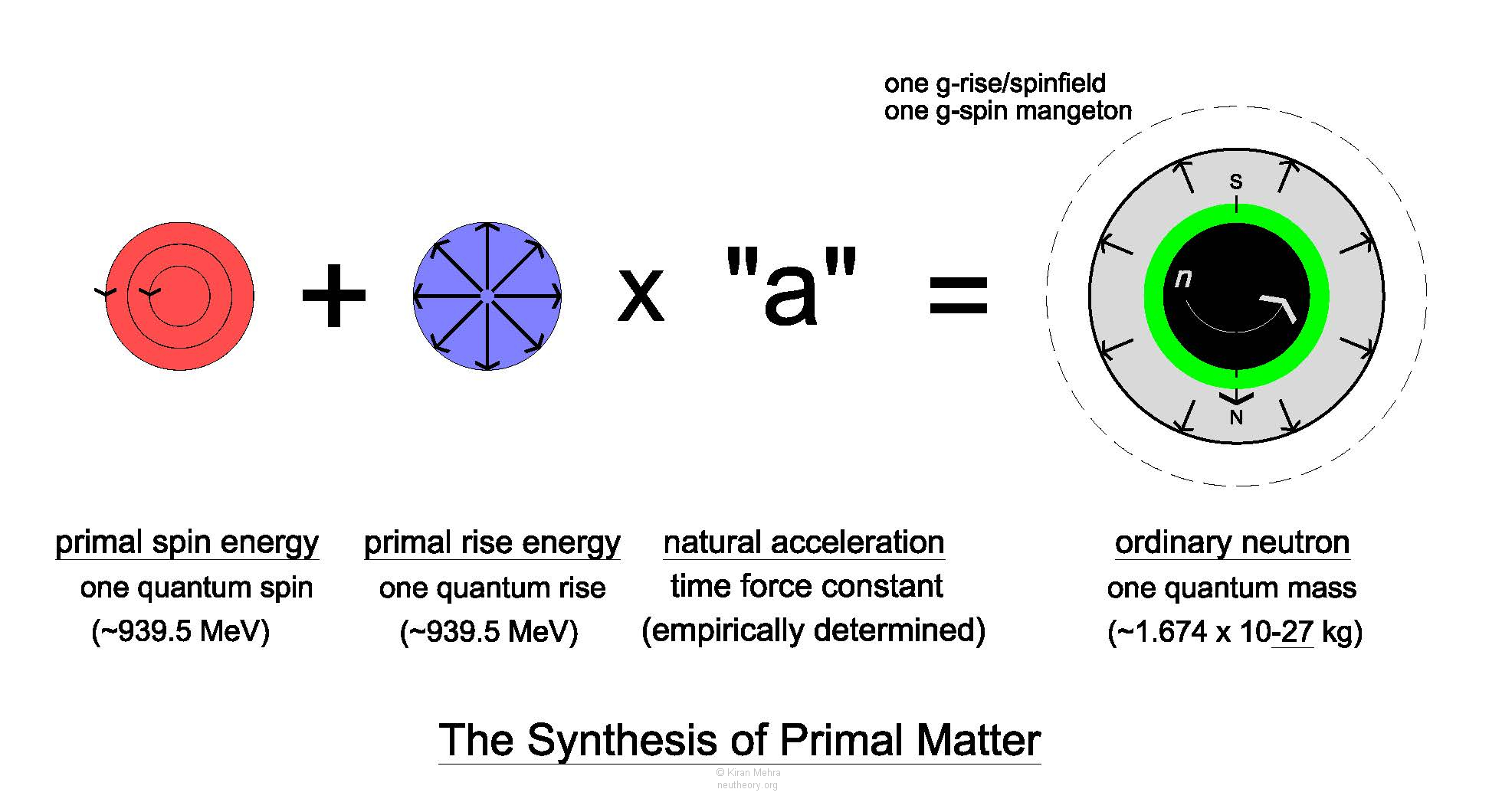Synthesis of Primal Matter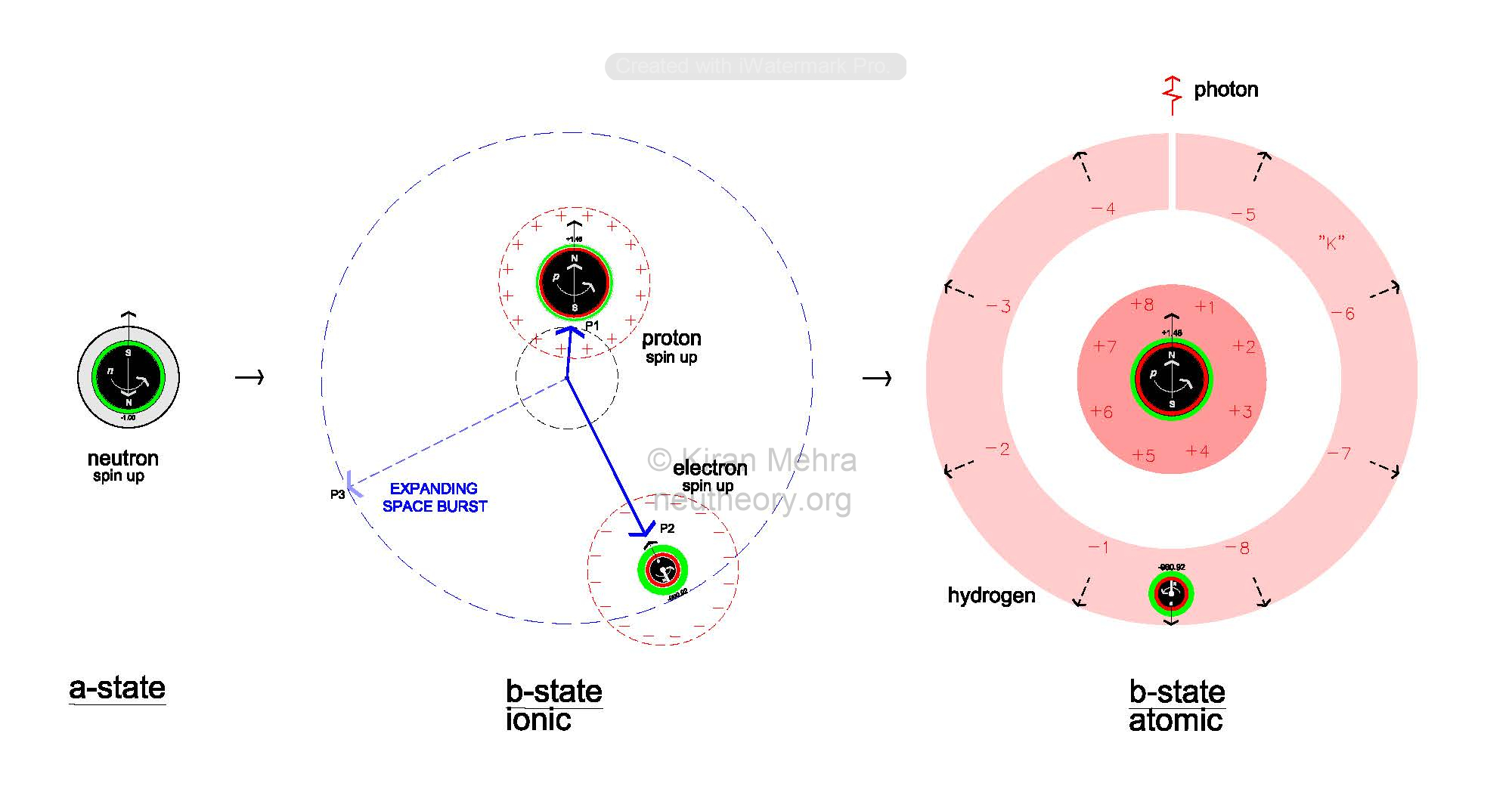Little Bang to Hydrogen Transformation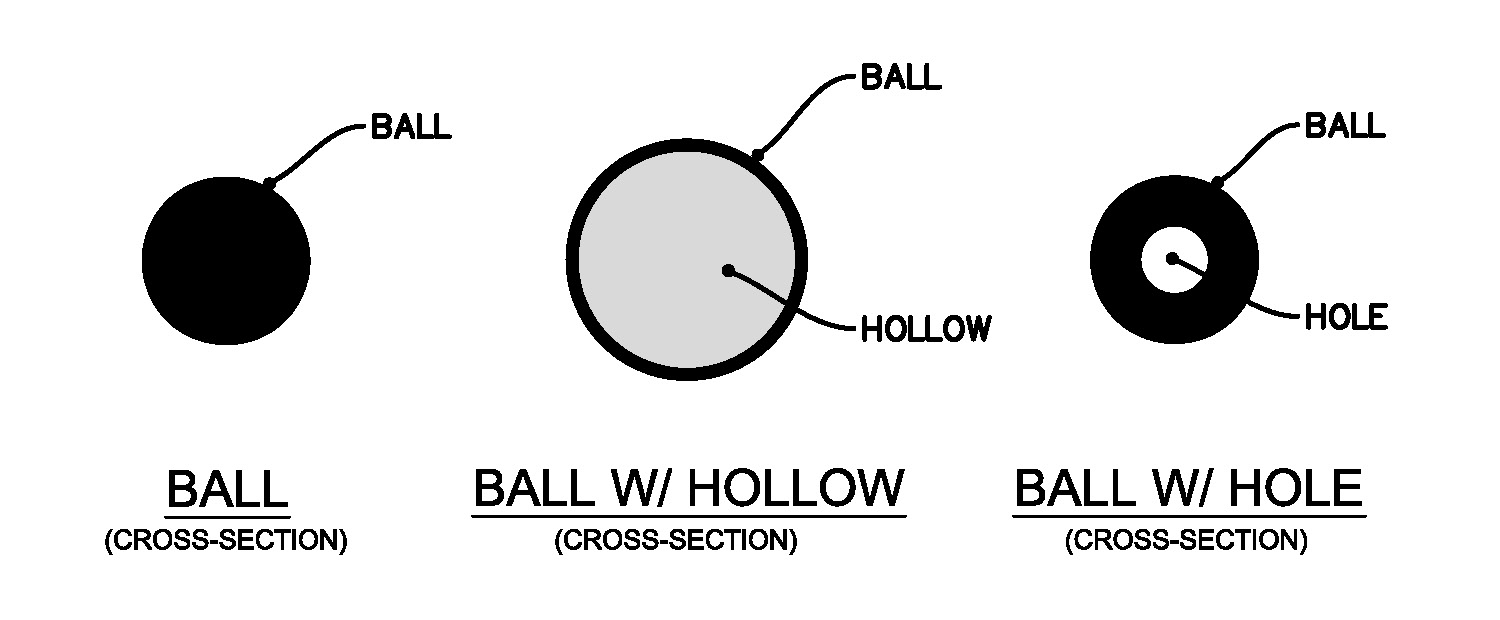Three Primal Shapes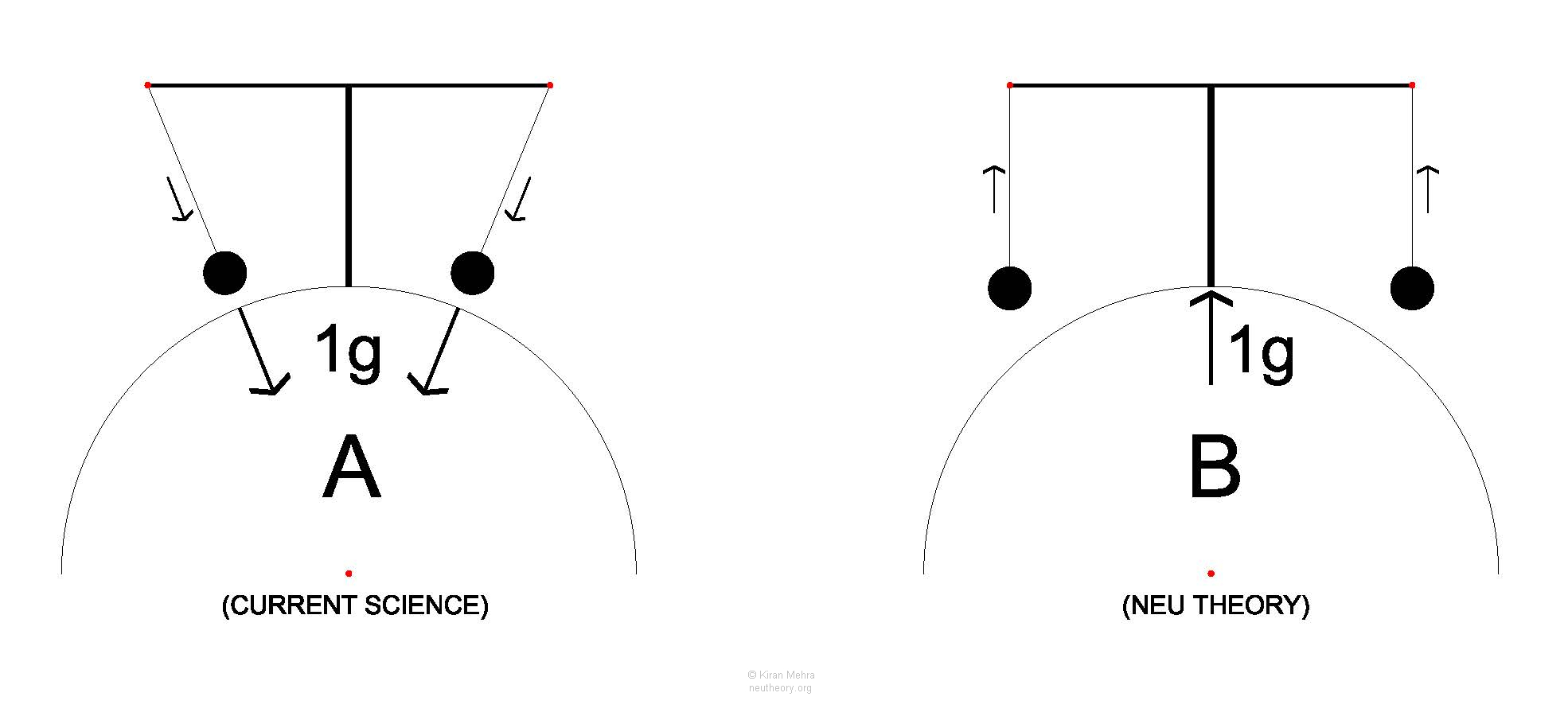Direction of Force – The Cantilevered Pendulums Experiment

The Seven Elementary Particles*

1. neutron matter core  (future proton core)
2. neutron matter membrane  (future electron ball)
3. neutron matter plasm  (future environmental energies of     electricity , space , motion  and light )
4. proton charge shell [6+] (positive, anode)
5. electron charge shell [6-](negative, cathode)
6. zomon  (quantum burst of space energy)
7. photon  (quantum bubble of light)

*[#] indicates fundamental form of nature [1of 11]

The Seven Primary Objects*

1. neutron cell 
2. proton [6+7+]
3. electron [6-7-]
4. deuteron cell [6+][7+]
5. helion cell [6+][6+][7++]
6. zome ∑  (cosmic space as nature’s largest object)
7. photon bubble  (radiant spectrum)

The Four Natural Accelerations*

1. g-spin of matter  (magnetic fields, spin alignment)
2. g-rise of matter  (push, spinfields, hyper-spinfields)
3. g-fall of charge [6,7] (compression shells, electric field)
4. g-rise of space  (space pressure, inertial field)

The Two Types of Matter

There are only two kinds of matter in nature, the model calls Type I Matter and Type II Matter.

Type I Matter is a neutral topologically homogeneous substance with a fixed absolute density at 6.693 x 1017 kg/m3. This density is empirically determined by dividing the proton mass by its volume as measured by the proton charge radius. If these values change the absolute density value will adjust.

The neutron core, the neutron membrane, the proton core, the electron ball, and the positron are Type I matter. Proton fragments made during high speed collisions are also Type I matter.

Type II Matter is a neutral topologically homogeneous substance with a variable density. The neutron plasm is the only form of Type II matter in nature and it can only exist as a quantum part of a neutron.

The difference between the least and the greatest neutron plasm matter densities spans an amazing 24 orders of magnitude.

The densest plasm is with Lead-208 at 9.68×1014 kg/m3. A one centimeter cube of plasm at this density would have a mass of 290 Empire State Buildings.

The least dense plasm is with an ultra cold neutron (UCN) at around 3.33 x10-10 kg/m3. A one centimeter cube of plasm at this density would contain approximately three one millionth (0.000 003) of a gram of matter.

The Two Types of Energy

There are two types of energy in nature, the model calls 1st Law Energy and 2nd Law Energy.
1st Law Energy follows the 1st Law of Thermodynamics.
2nd Law Energy follows the 2nd Law of Thermodynamics.

1st Law Energy is a form of energy that accelerates with time and is not subject to entropy. The free spin energy of the electric dipole charge shells and the free rise energy of space are the two forms of 1st law energy in nature.

2nd Law Energy is a form of energy that does not accelerate with time and thus becomes subject to natural entropy. The bound rise energy with the kinetic motion of matter, and the free spin energy of light are the two forms of 2nd law energy in nature.

Spin Energy and Inertia

A good question is why spin energy has not been recognized by science as a separate form of energy. There are perhaps several reasons:
1. Almost all of the spin energy in nature, over 99.7%, is linked together with rise energy as matter and not available to be directly examined as a separate entity.
2. All of the spin energy (0.7827 MeV), delinked from quantum plasm matter with the little bang becomes the mirror-split free spin energy of the discrete equal and opposite (positive and negative) electric charge shells that surround the proton and electron matter couple.
3. 100% of primal quantum spin energy remains with matter and the electric charge shells, none goes anywhere else.
4. Historically, electric charge has been considered to be an intrinsic property of some forms of matter, not a discrete form of energy. Even the fractional 2/3+ and 1/3- charges of the “quarks” of current science are kept well “inside” the proton where they cannot be directly examined.
5. It is hypothesized that spin energy, half of nature’s total energy, carries no inertia. Both the 1st law free spin energy of the electric charge shells, and the 2nd law free spin energy of the photon have no mass.
6. It is hypothesized that all the mass (inertia) comes from the rise energy content of matter and space only. Remarkably, spin energy is what binds and holds matter together, giving it its shape and size, yet adds no “weight” to the union!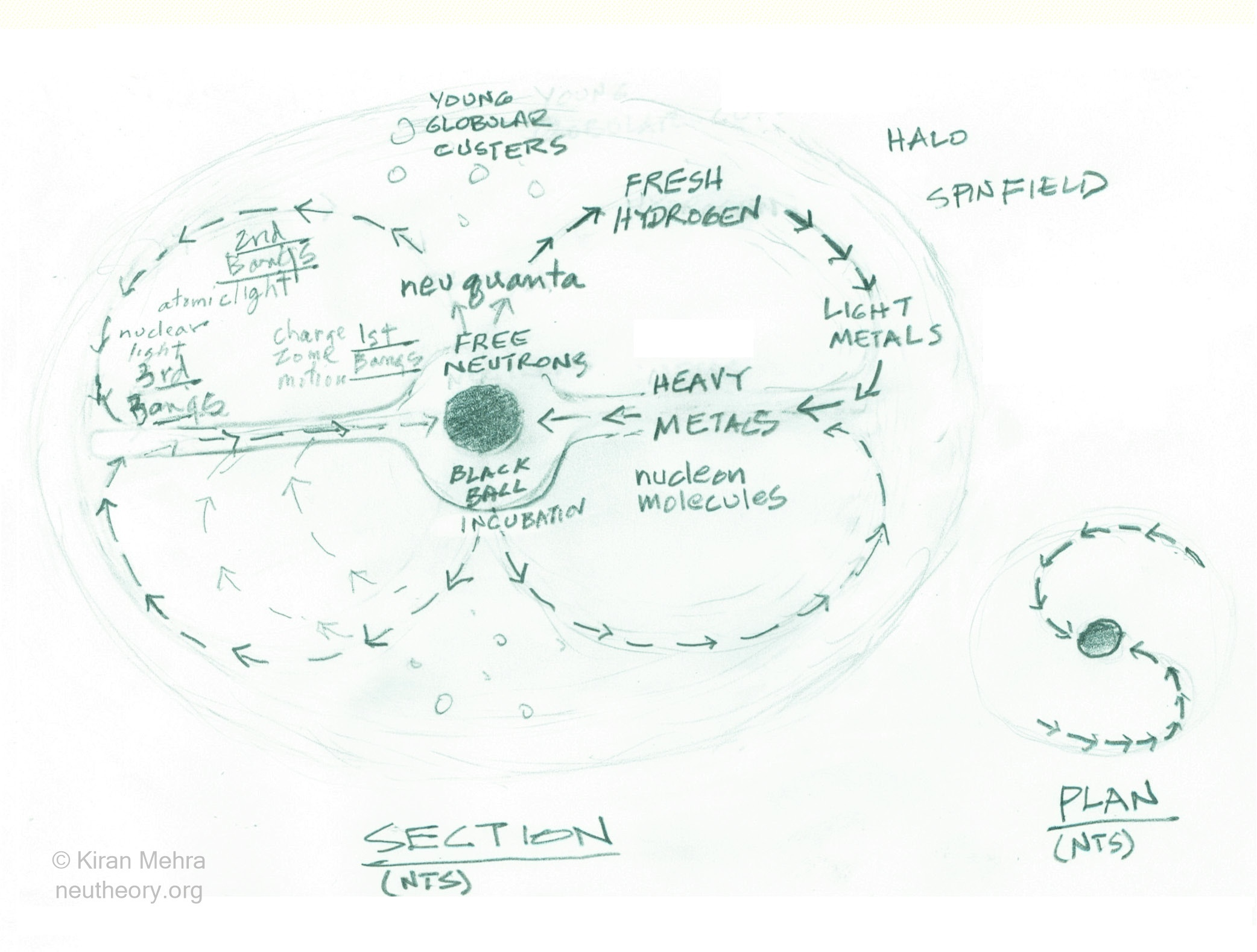The Spiral Galaxy Form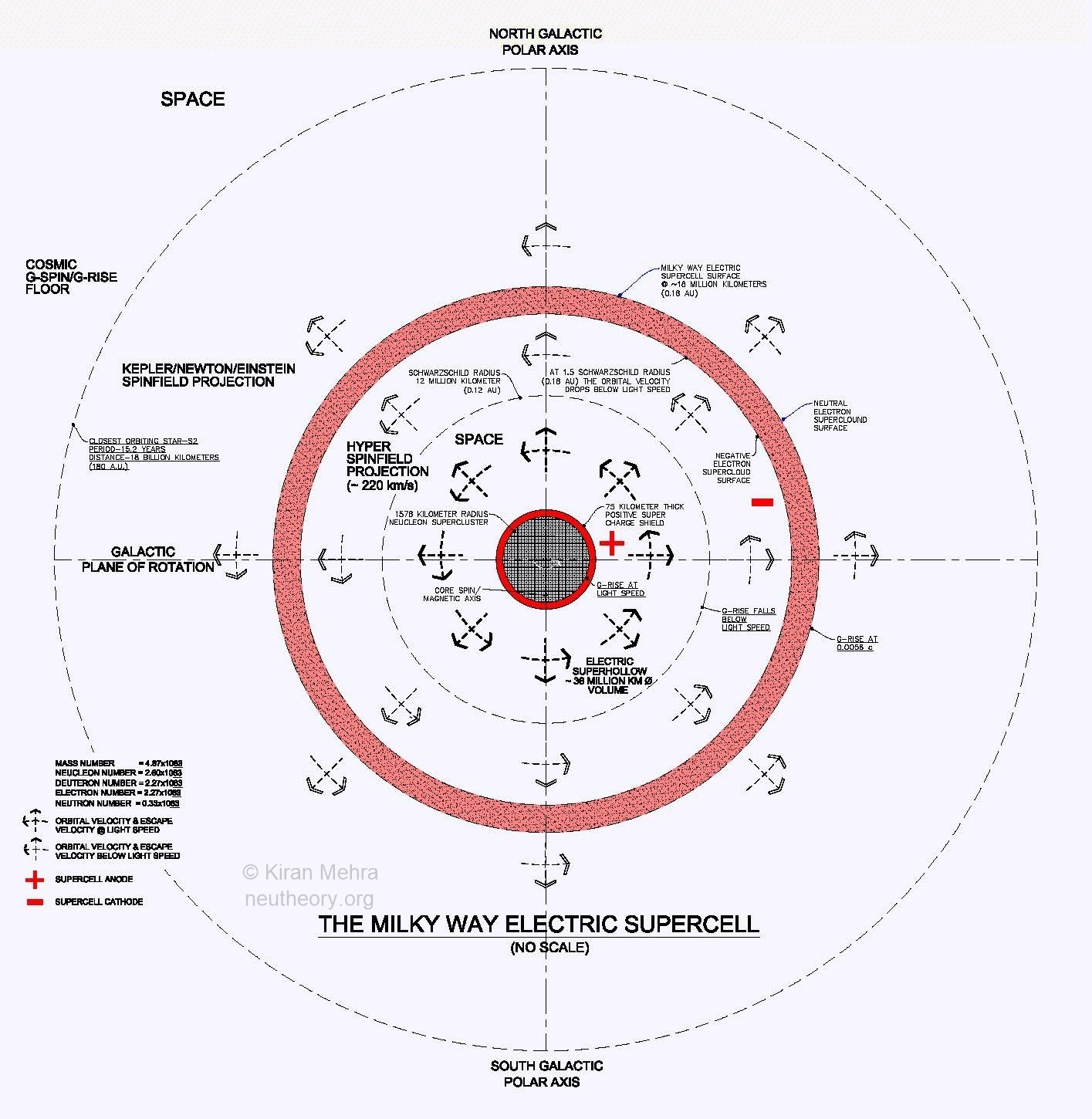The Milky Way Electric Supercell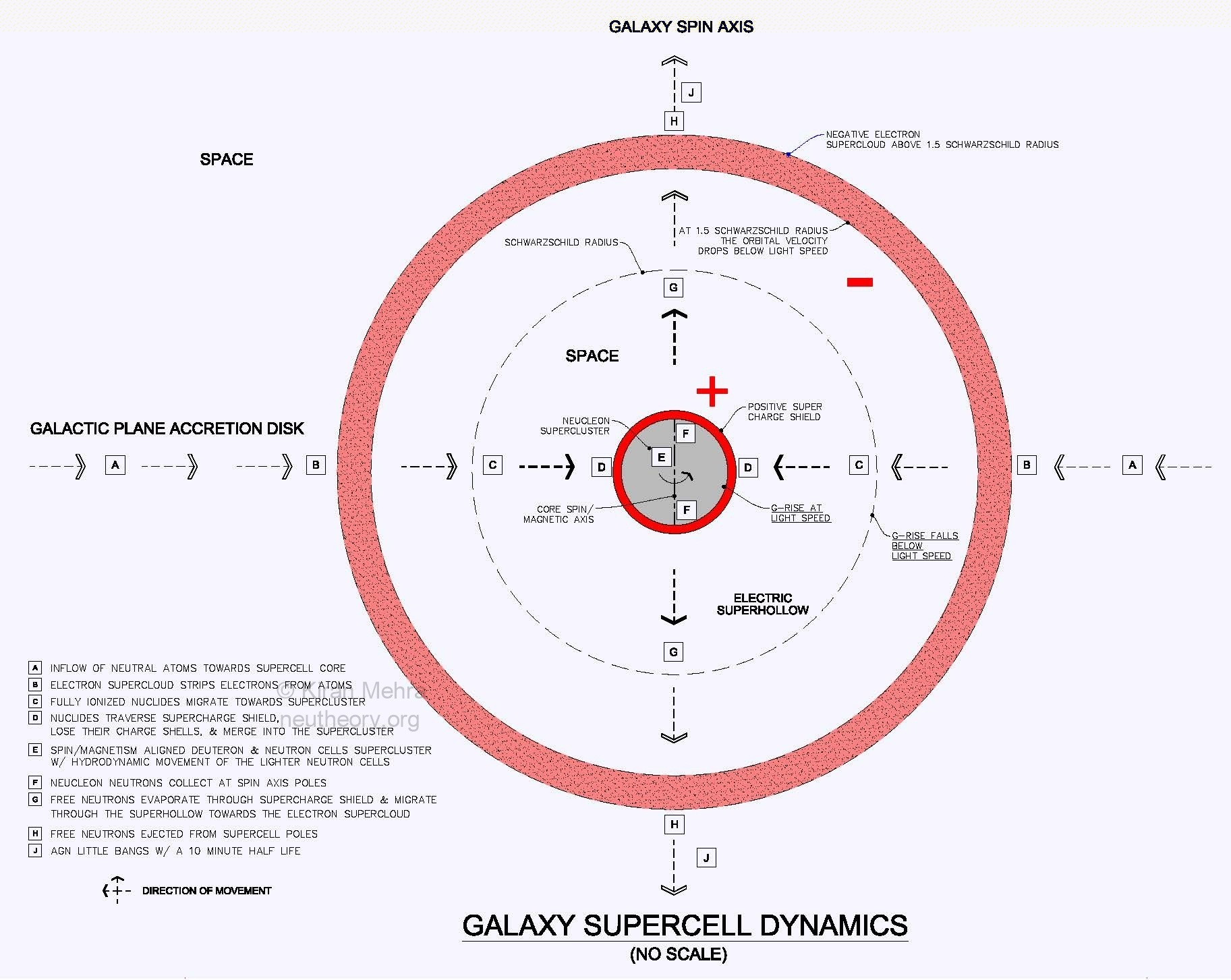Galaxy Electric Supercell Dynamics (Black Hole)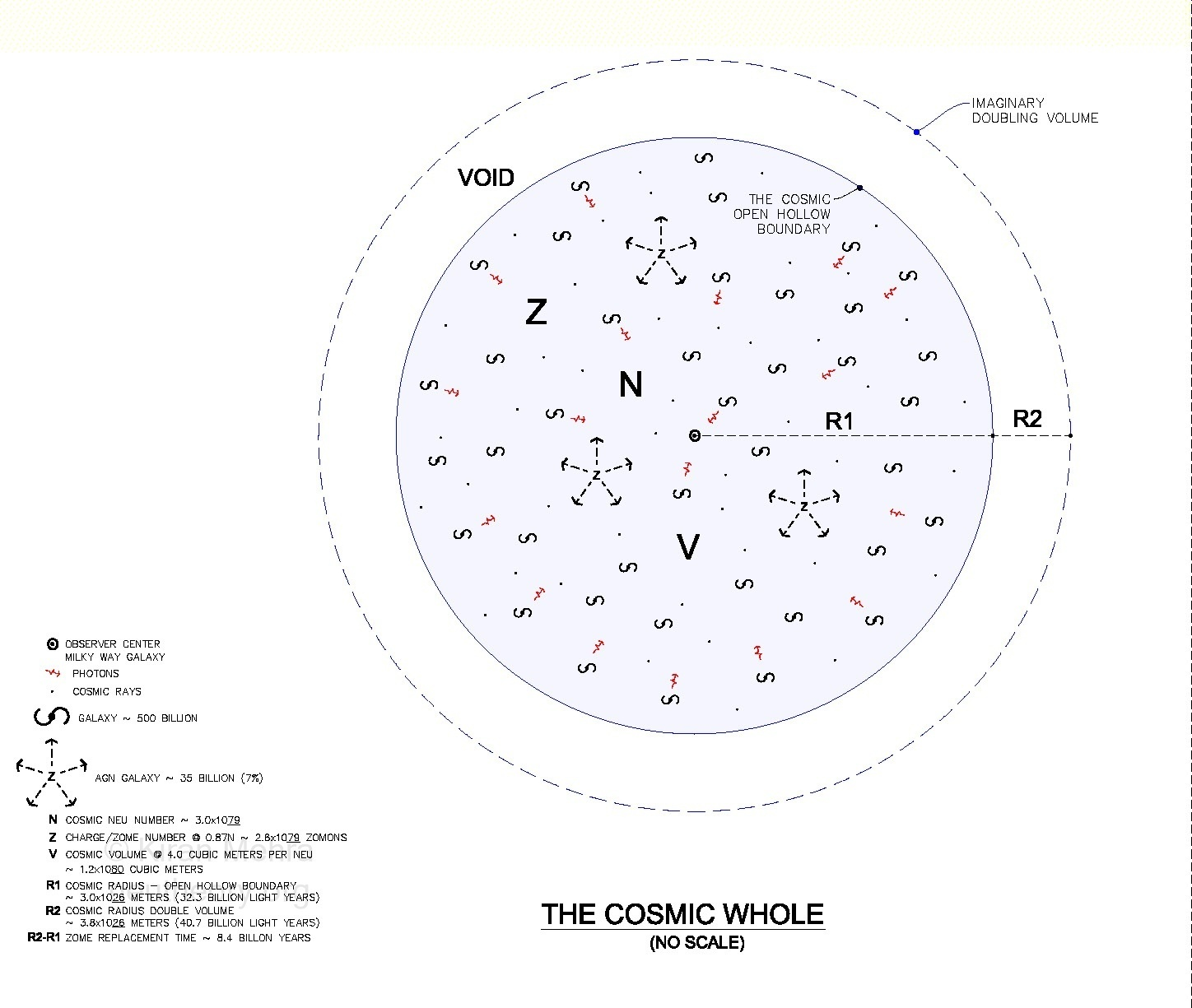The Cosmic Whole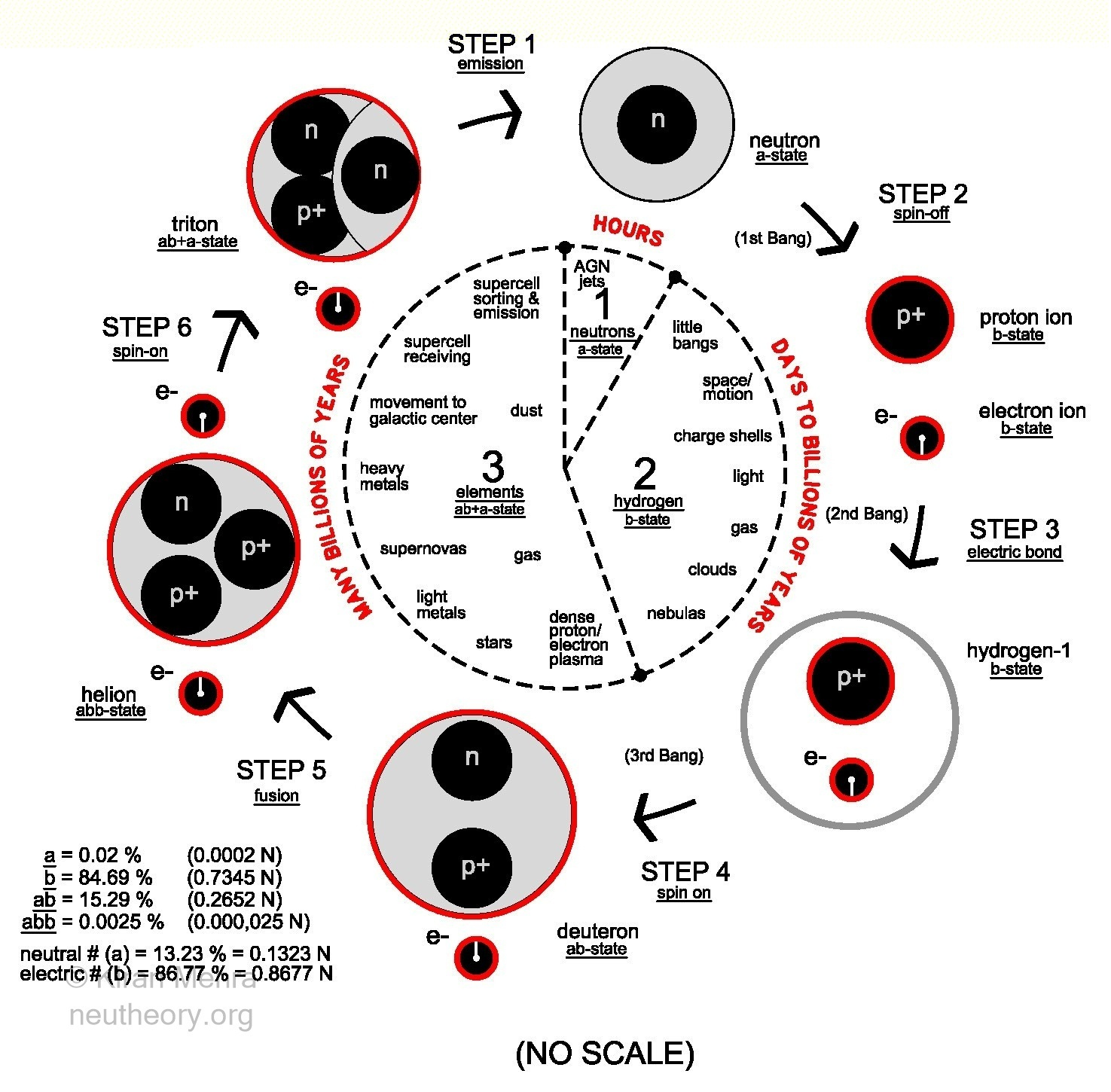The Atomic Matter/Energy Cycle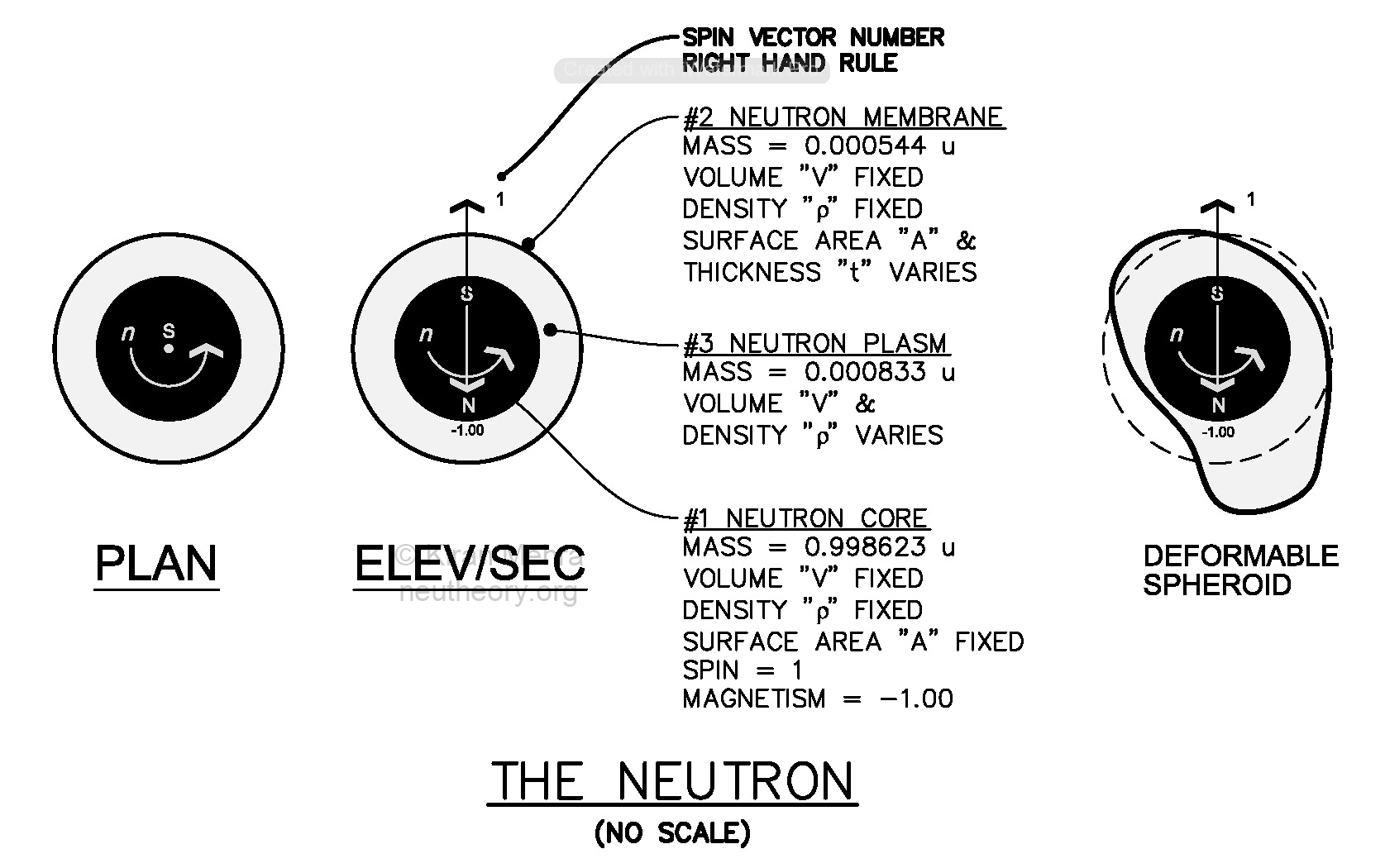The Neutron Cell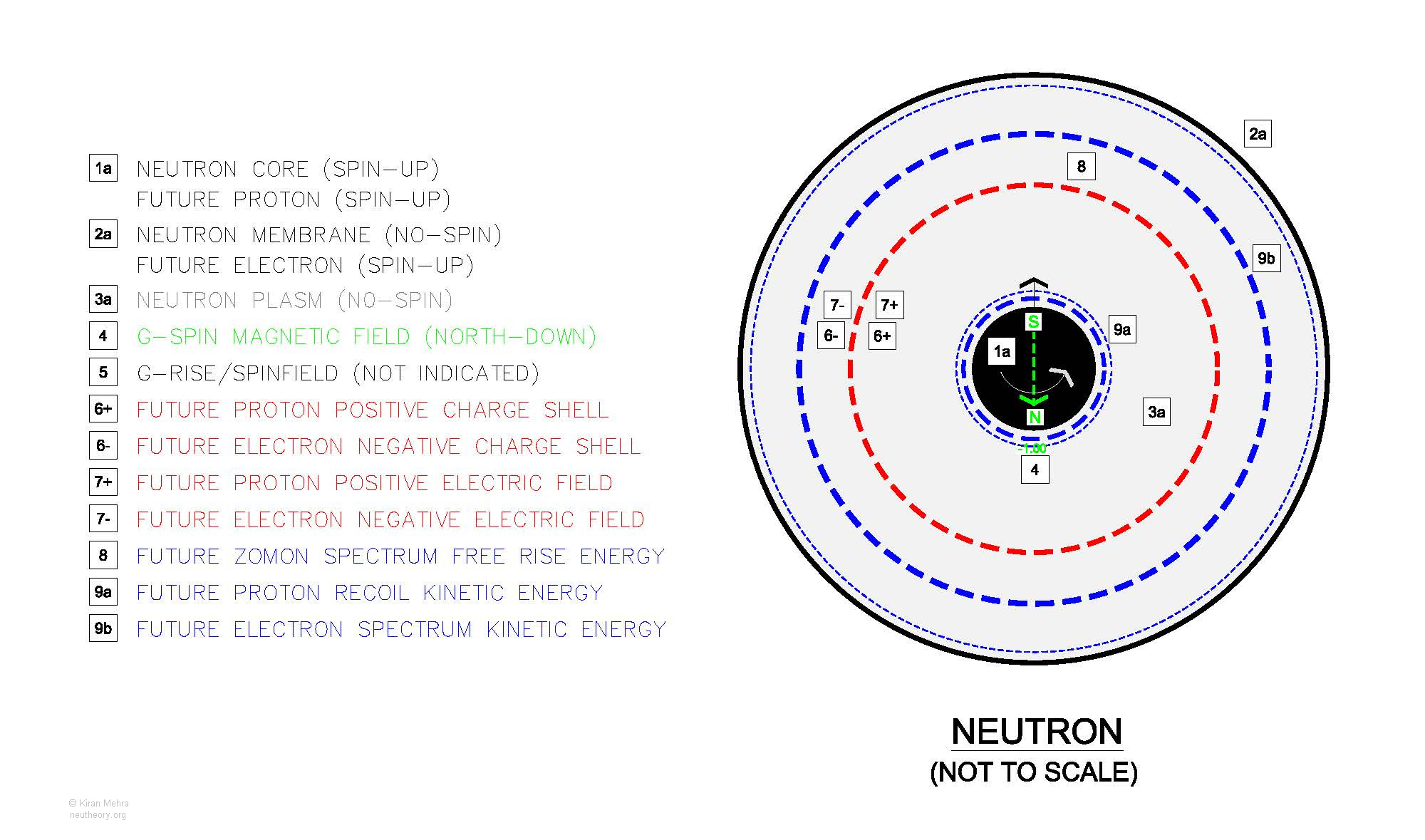Neutron Cell Pre-Transformation Topology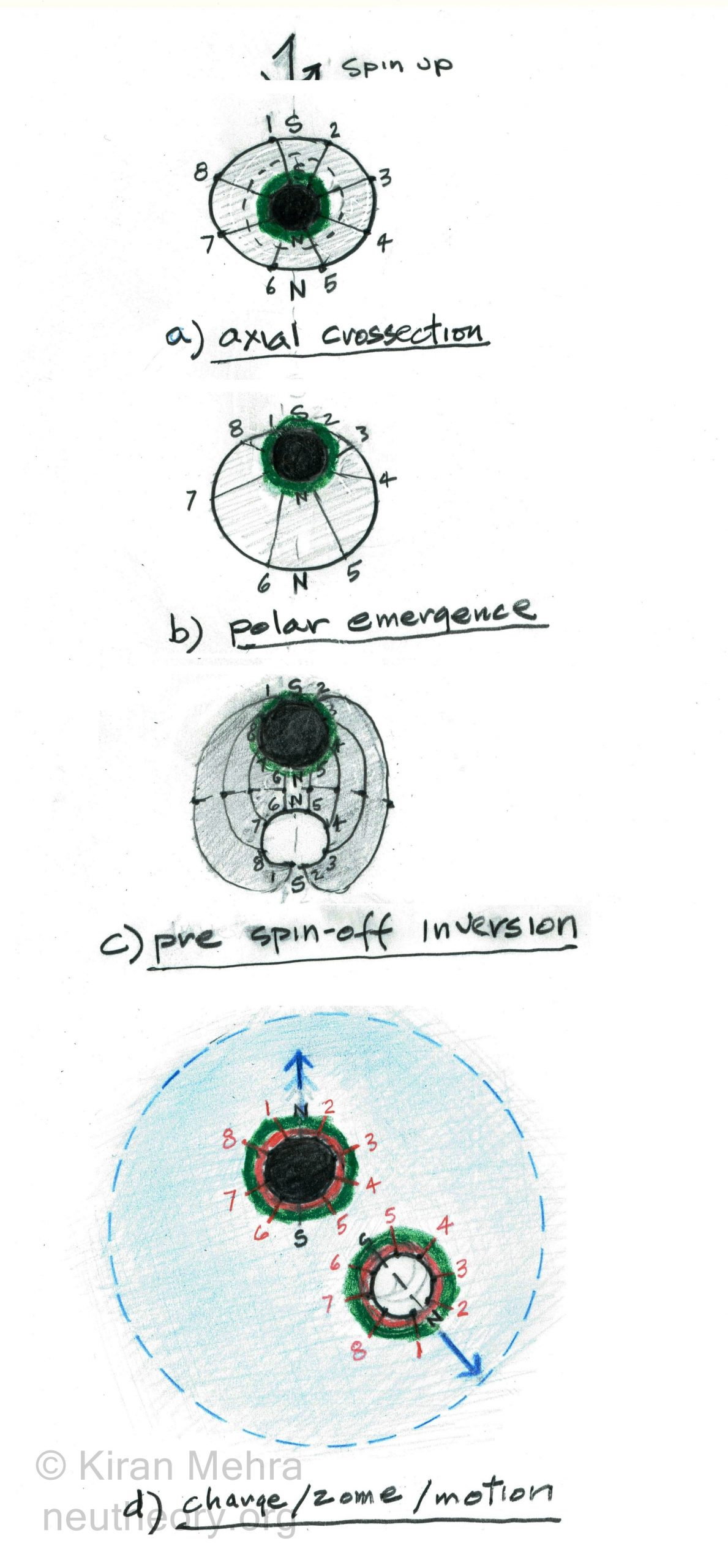The Little Bang – Spontaneous Neutron Transformation Sequence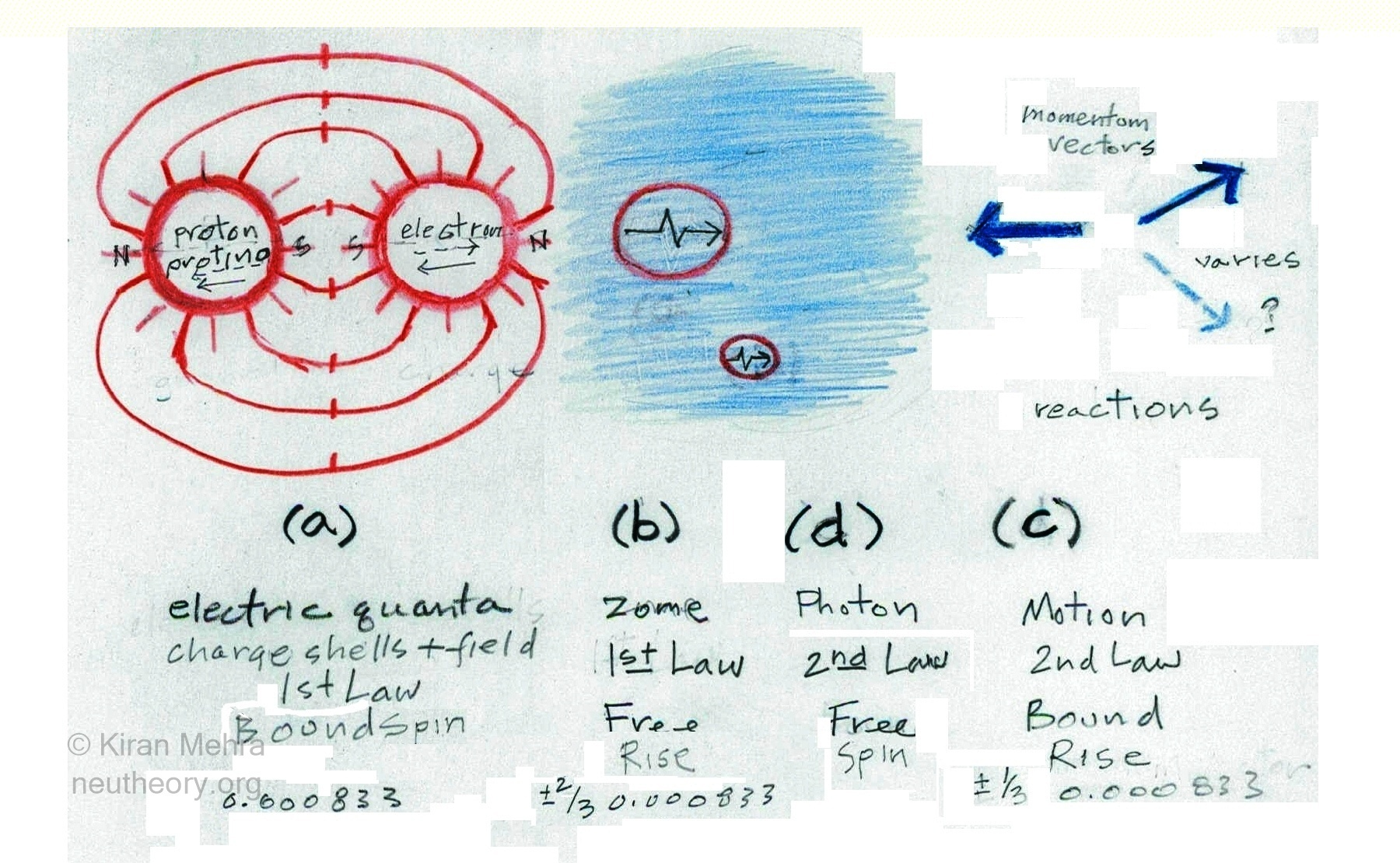The Four Energies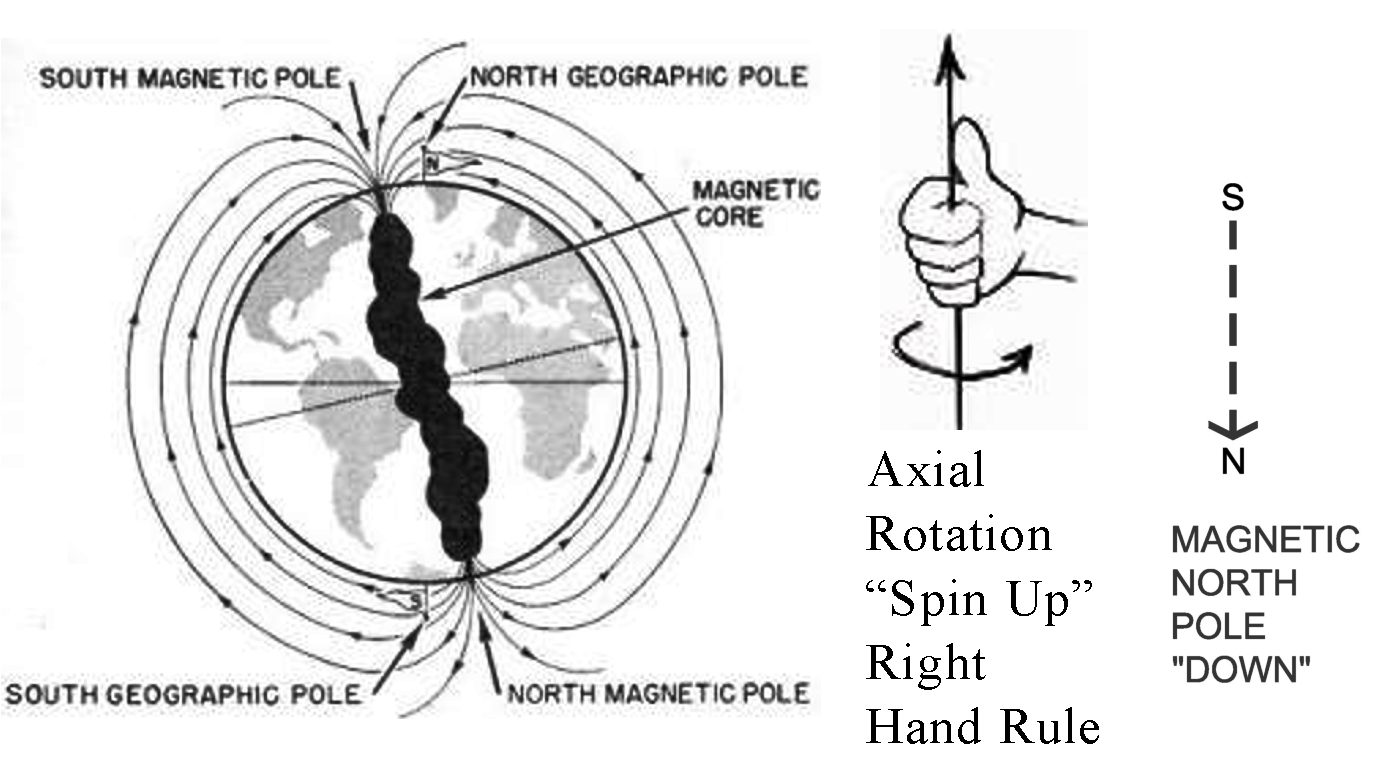Spin/Magnetic Orientation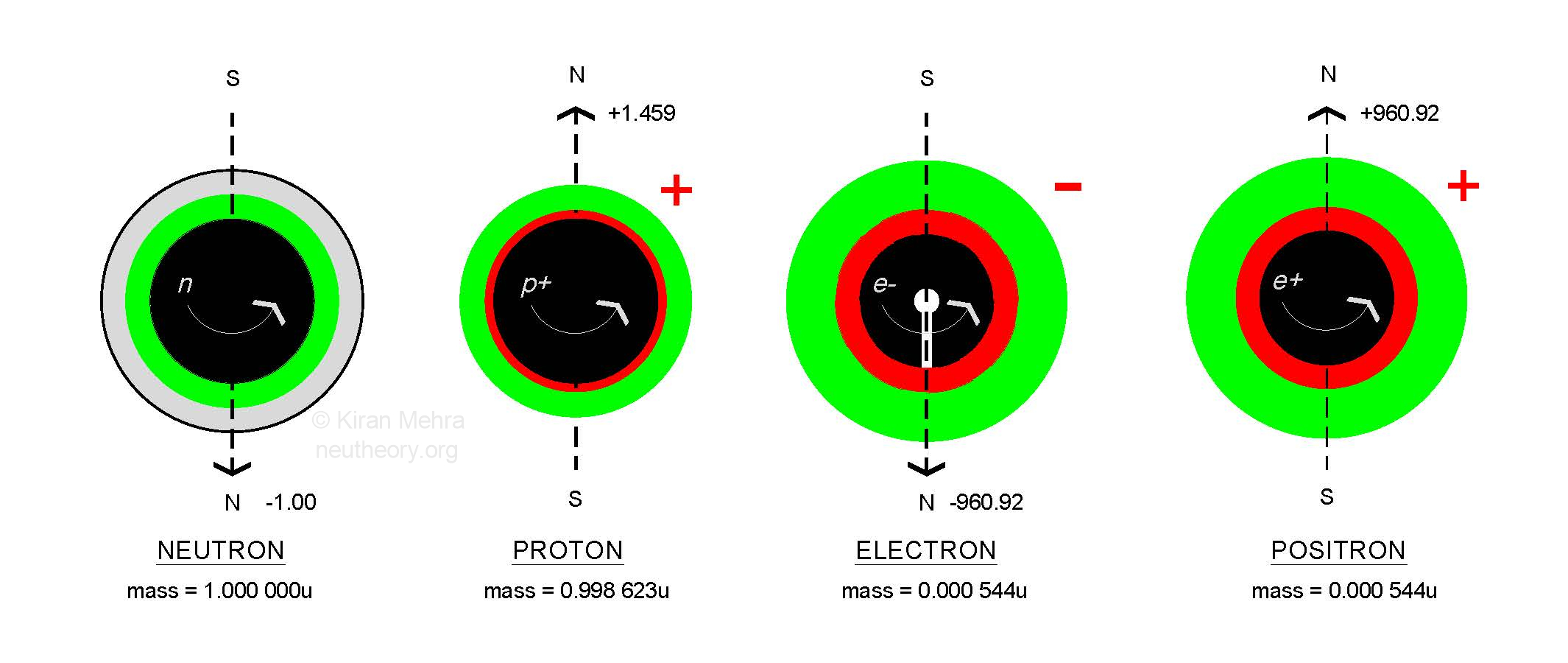Primary Spin/Magnetism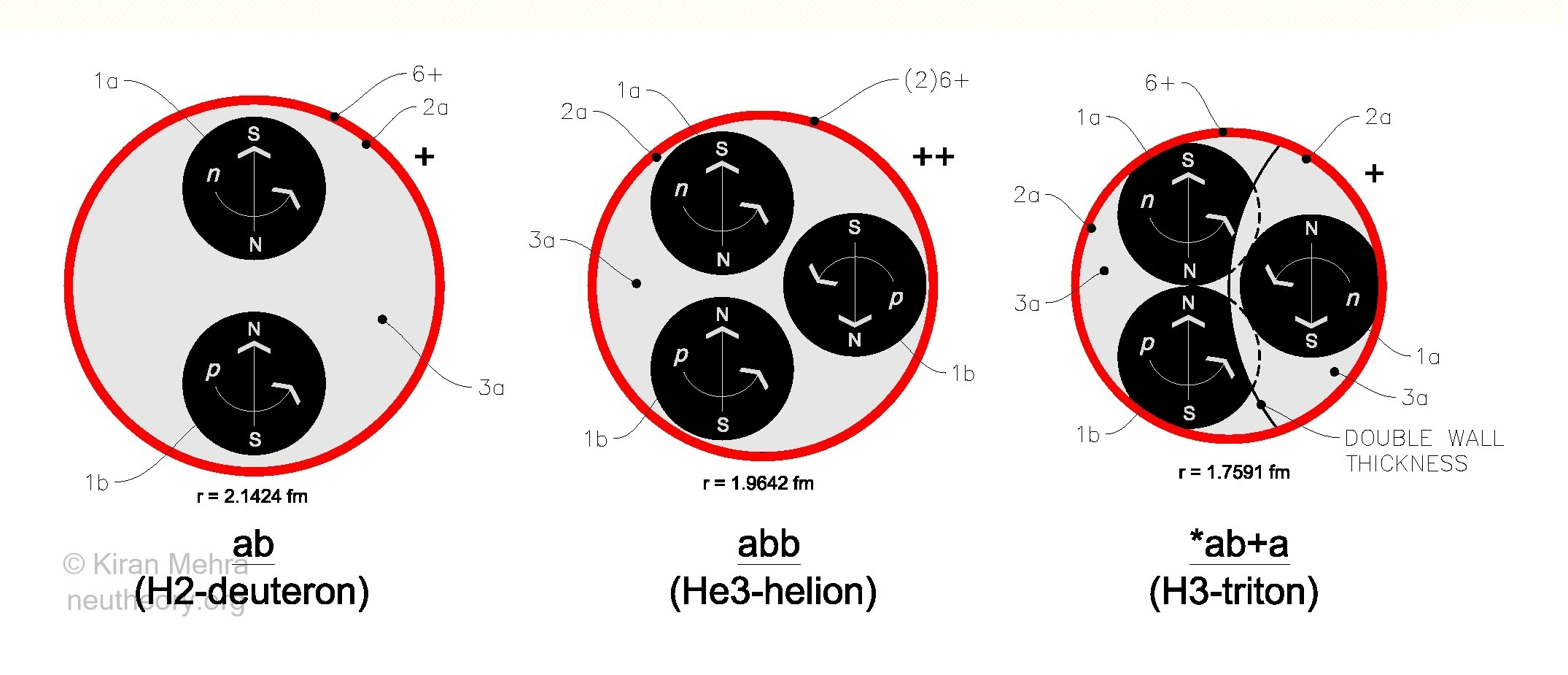The Three Neucleons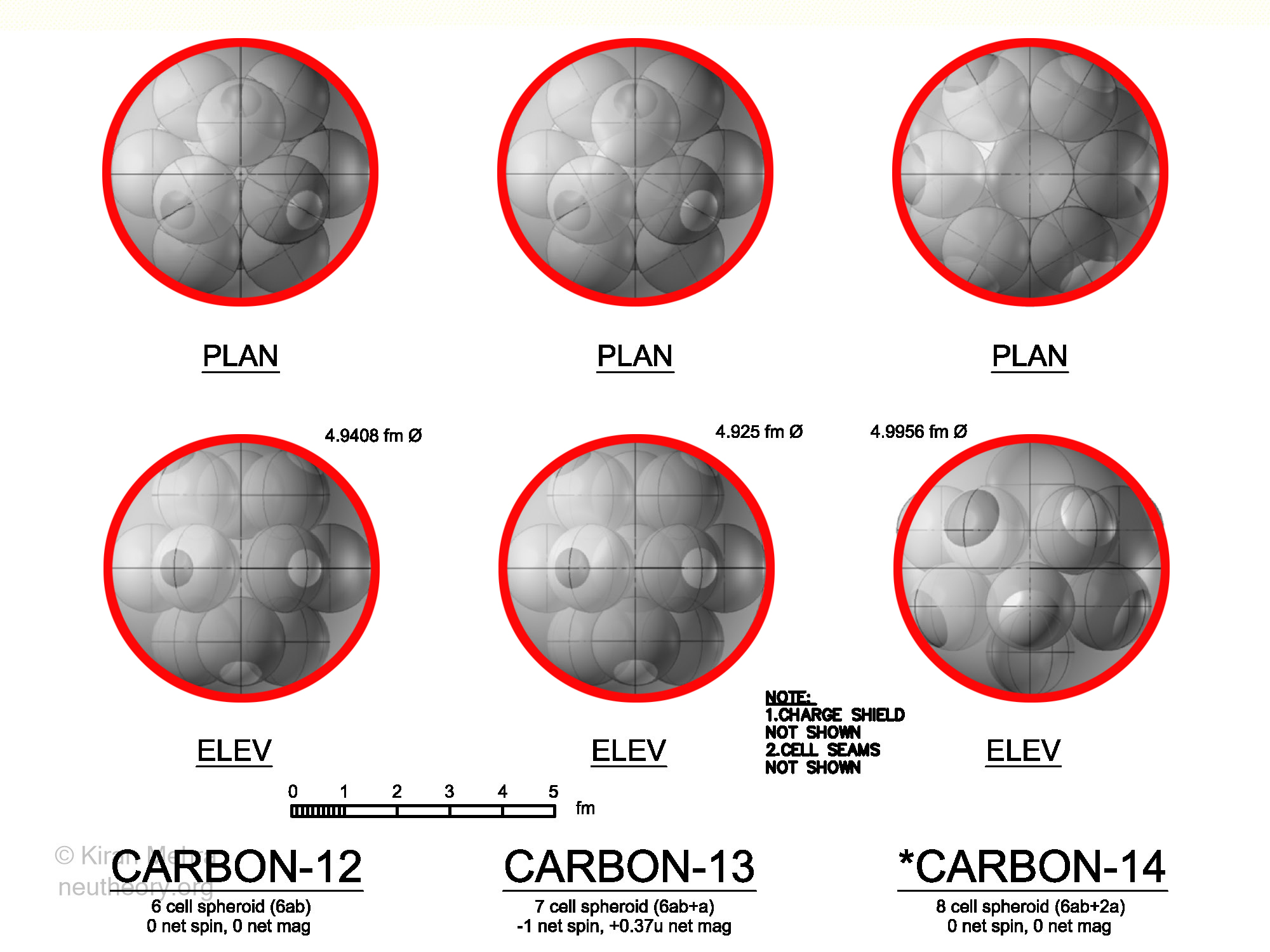Carbon IsotopesNitrogen Isotopes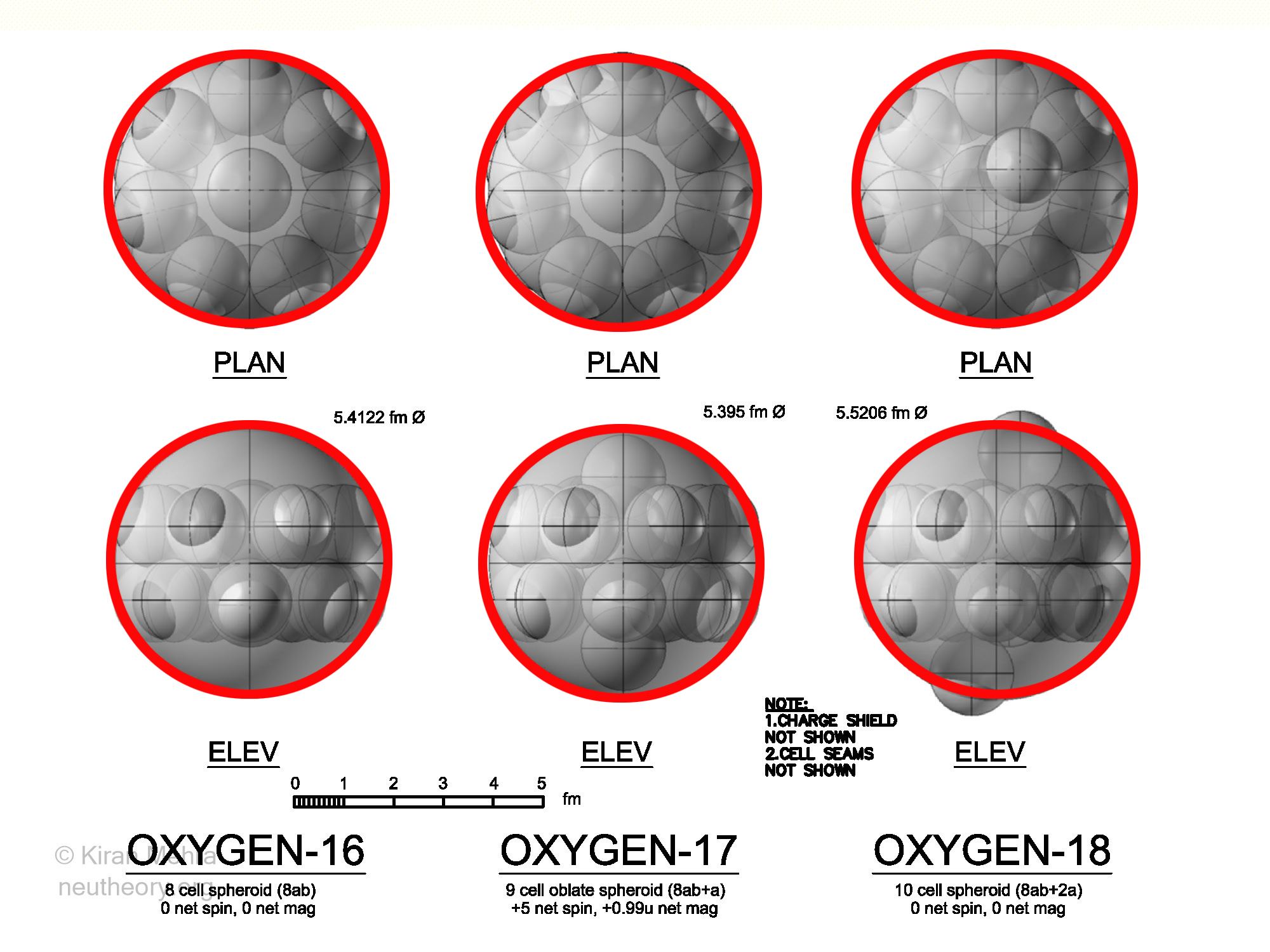Oxygen Isotopes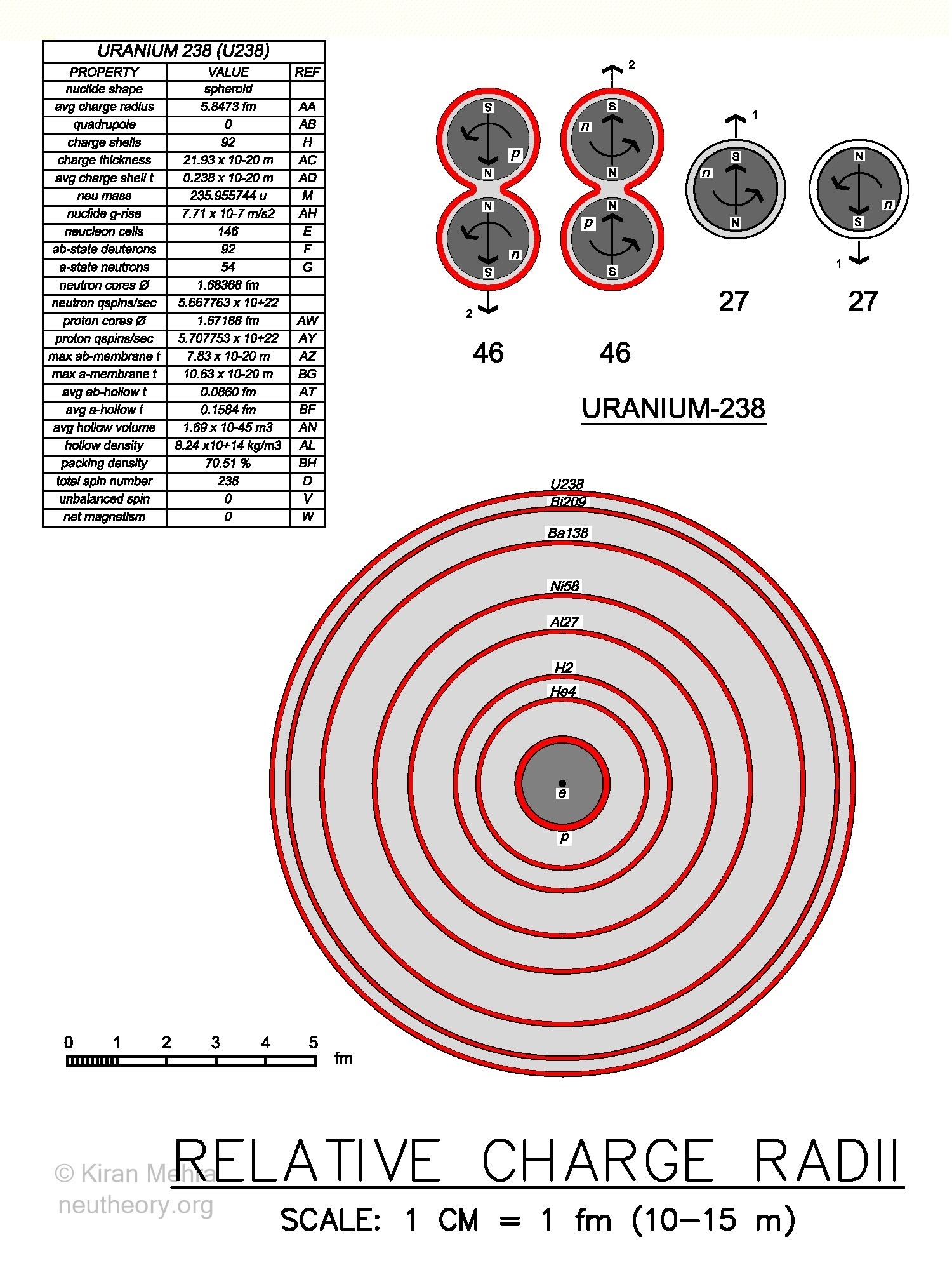Relative Charge Radii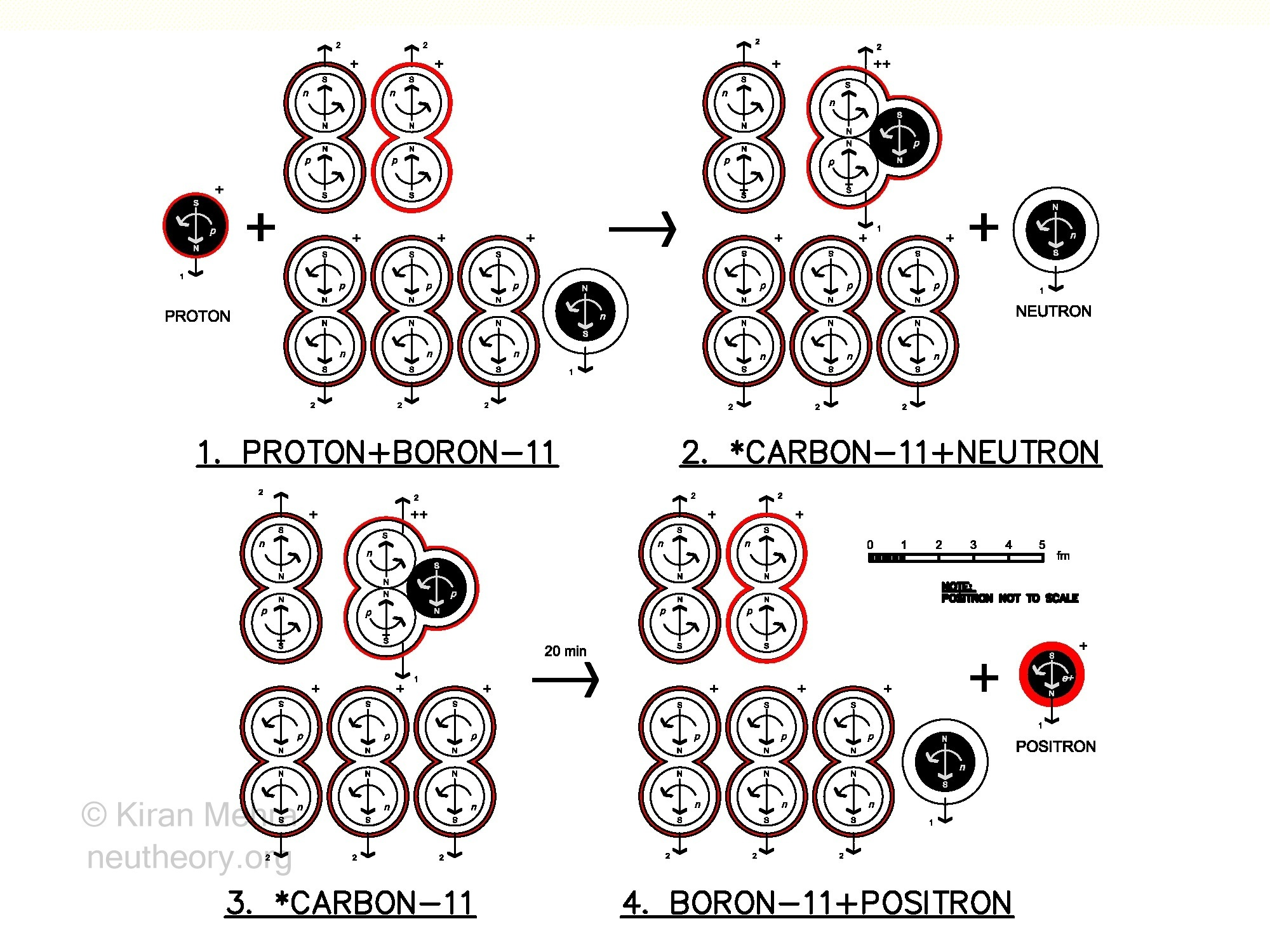Positron Emission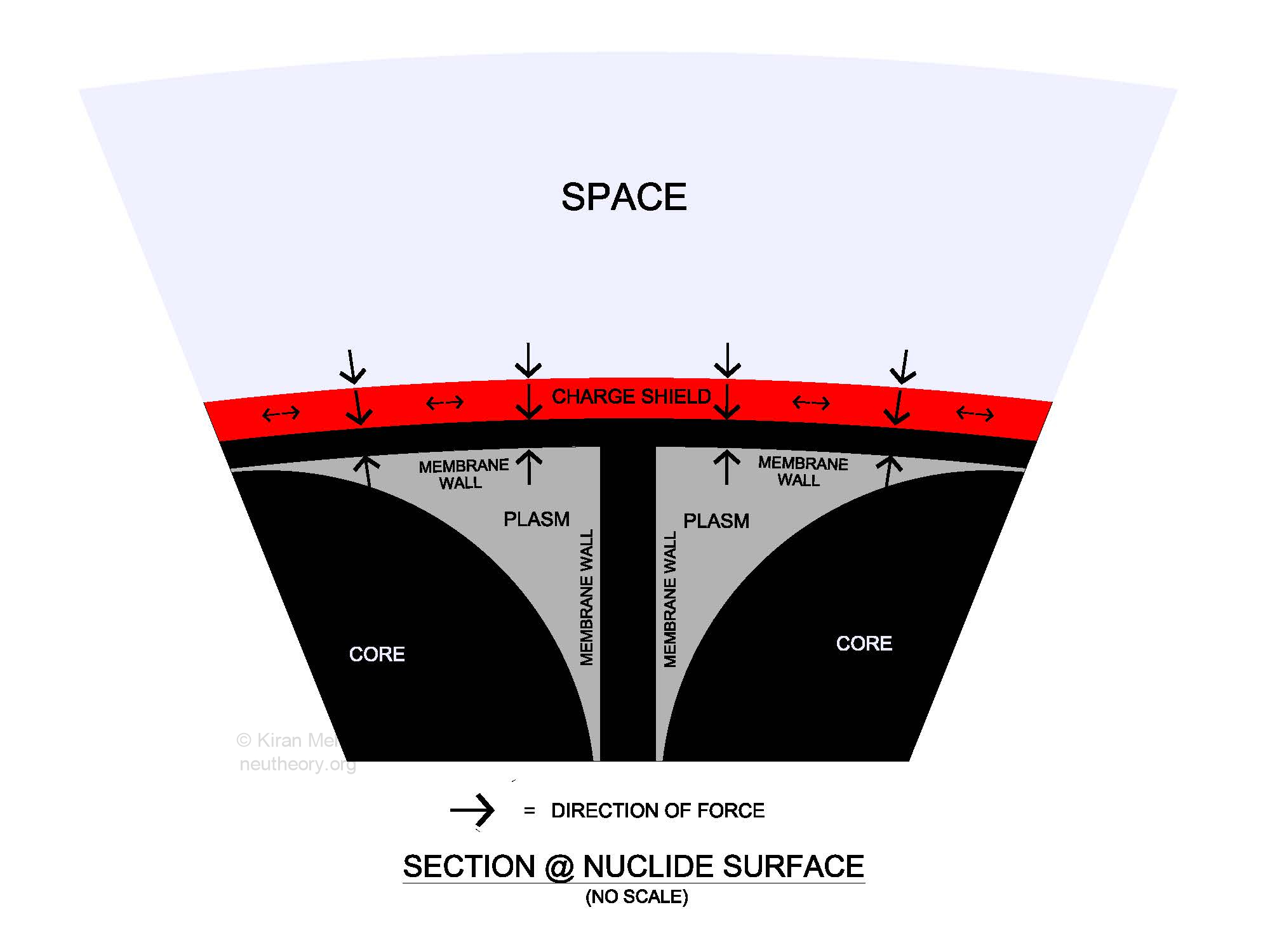Section @ Nuclide Surface

Unbalanced Force in Nature

In Neu Theory, there are no unbalanced attractive forces in nature.

Both spin/magnetic dipoles and electric charge dipoles, attract and repel, thus able to neutralize, or balance each other creating a net zero effect. Both magnetism and electricity are balanced forces of nature with a limited effective range.

However, both Newton’s universal law of gravitation, and the strong nuclear force that is required to hold the nucleus together, are unbalanced attractive forces.

Since Newton, gravity has been hypothesized as a weak universal force of attraction between matter, that has a unlimited range. The force of gravity can only add becoming unstoppable when matter “falls” below its Schwarzschild volume becoming a “black Hole.” Here it is speculated that the matter shrinks into a “singularity,” a place without volume, where the ordinary laws of physics do not apply. Anything can be hypothesized within such a place. There are no rules. Without an initial singularity, there cannot be a big bang

The strong nuclear force is hypothesized by current science as a very strong attractive force, one hundred times stronger than the electric force, that nature provides to hold the protons and neutrons in a nucleus together. Otherwise, it is believed that the strong repulsion between the positively charged protons would blow the nucleus apart. This force has a very short effective distance, decreasing faster than the usual inverse square of distance.

In Neu Theory there is only one unbalanced force in nature and there is nothing attractive about it. This primal force is the universal time/force acceleration constant a. The constant a is a perpetual one-way input of force, a constant natural pressure, that acts through matter and energy forms generating the the four g-forces that power the cosmos.

G-Rise/Spinfield (Gravity)

The g-rise/spinfield is gravity in Neu Theory. This form of nature [#5 of only 11 fundamental physical forms] consists of two parts that act together and cannot be separated from each other.

The first part of gravity is g-rise, the natural acceleration-in-place of matter that, similar to Newton’s gravity, varies directly with mass and inversely with volume. All three volumes of primal matter (core, membrane, plasm) are subject to a perpetual internal acceleration from the natural constant a. This acceleration which can be felt but not seen, is the phenomenon we call gravity. On a practical level, this acceleration-in-place starts at the surface of protons, electrons, and nuclides. As the numbers add, the objects they make have a combined acceleration-in-place that can only add.

With a neu number of two, the deuteron (Hydrogen-2), has a calculated g-rise at the nuclide surface equal to 0.49×10-7 m/s2, the smallest of any atom. With a neu number of 208, as with the isotope Lead 208, the g-rise at the nuclide surface is 7.61×10-7 m/s2, the largest of any atom.

With the Earth neu number (3.57×1051) and volume, the g-rise acceleration-in-place at the surface is 9.8 m/s2. We feel this as a continuous push to our bodies with the occasional bump, or worse, when we fall down. We can measure this force, calling it one g of gravity.

The g-rise of a white dwarf star, about the same size as the earth but with a much larger mass, is approximately 300,000 g.

With larger neu numbers, starting at around 3 solar mass (3.56×1057), a violent neucleon collapse can occur where all the individual atomic nuclides fuse into one giant atomic nucleus with one charge shield. This is an electric supercell or black hole, where the surface g-rise acceleration in place of the core ball has reached the speed of light c, maxed out, and stays there.

The second part of gravity are the spinfields. The g-rise spinfield takes two forms.

1. The first spinfield form is the spinfield generated by the g-rise of matter at speeds less than the speed of light c.

2. The second form is the hyper-spinfield generated by the g-rise of matter at the speed of light c, as in a supercell core.

The g-rise of matter, projects a movement/synergy field into space that is experienced by other bodies. The trajectory of objects that come inside the object’s spinfield volume, are subject to predictable changes in their direction and speed.

G-rise  at speeds less than c generates a spinfield with the same practical effect as Kepler’s laws of planetary motion, Newton’s gravity, and the “spacetime” of Einstein’s General Relativity Theory. The formulas and equations always works. Nothing is being changed here.

The crucial difference between Neu Theory and current science, is the nature of space and time itself. More like Newton than Einstein.

G-rise at c is hypothesized to generates a hyper-spinfield with a different, yet to be determined, physics. The practical effect is a uniform orbital speed for satellites surrounding the hyper-spinfield generating body.

In General Relativity spacetime is the “bending” or “warping” of space itself by the presence of mass. The greater the mass contained within a unit volume the larger the warp and slower the flow of time.

In Neu Theory the g-rise/spinfields, like every other field in nature, e.g., magnetism and electricity, is a movement synergy projection into space, that does not change the underlying properties of space itself.

In this model, space is a maintained homogeneous volume consisting of ~2.6×1079 free rise energy quanta that are expanding and diffusing-in-place at the accelerating speed of light. Rise energy is a permanent “straight” one-way movement that cannot be changed. Space never bends, and the flow of time is the natural acceleration constant a that never changes.

Magnetism and Electricity in Neu Theory

In the Neu Theory model, magnetism only exists with the g-spin of elementary particles, and is discrete from electric charge.

This is different than the current scientific paradigm, that magnetism is ultimately created by some electric charge, or fractional charges, that reside within the body of an object. It is believed, that first there has to be some electric charge, only then can magnetism appear. Hence the current view, that “electromagnetism” is one fundamental force of nature.

The Neu Theory model identifies electricity as a spherically split quanta (0.7827 MeV) of free spin energy from plasm matter, that has been topologically “unzipped”, into equal and opposite “positive” and “negative” mirrored shells with equal volume and spin energy, that “jacket” the proton and electron. The discrete spin energy shells maintain isotropic closure around the neutral matter objects at the accelerating speed of light.

The primal neutron cell is completely neutral and is a magnetic dipole. In principle, there can be no electric charge (split isotropic spin energy) contained within any of the neutron’s three parts, as this would make it larger than a quantum unit. All scientific experiments performed to date searching for any electric charge within a neutron have failed. No indication of charge has ever been found and none ever will.

Space, Light and Redshift

Space is the perpetual isotropic expansion and diffusion-in-place of 1st law free rise energy at the accelerating speed of absolute movement.
Light in the form of photons are 2nd law free spin energy bubbles with isotropic closure at the speed of light that existed at their moment of birth. Photons do not accelerate with time. A photon’s time of closure (ct) is its size (wavelength) and its energy (color or frequency).
Photons do not move through space, they are carried by moving space in the unique direction of their birth. Photons are the largest number of objects in the universe, with an estimated several hundred million photons of all colors, traveling in all directions in every cubic meter of space. Photons are “bosonic” creatures and do not interact with each other, they only interact with matter and space. The interaction between photons and space comes at a cost for the photons. This cost is a loss of internal energy measured as “redshift.” Consider the following two causes:
1. Space pressure – The photon “bubble” uniformly increases in size (loses energy) during transport due to the uniform g-rise pressure of space energy acting from inside the bubble. This is the “Hubble” redshift based on the distance of travel, a “milage” tax. If you double the distance the redshift also doubles.
2. Space acceleration – Concurrently, in addition to the Hubble redshift the photon bubble increases in size at an accelerating rate based on its “age”. This is the “dark energy” redshift – a small compounding depreciation of photon energy based on the accumulated time of travel, the “aging” tax. If you double the time of travel this redshift is quadrupled, however as the uniform universal acceleration constant a is so small to begin with it takes a long time (several billion years of travel) before the energy loss begins to be significantly noticeable above the Hubble redshift. This “aging” redshift measures the rate of acceleration “a” of the current speed of light.

Space and Motion

The little bang is the birth of space and motion. Both are created from de-linked quantum plasm rise energy at the same time. Space is always moving at the accelerating speed of light perpendicular to matter and charge, however fast matter is moving relative to matter. The reason the speed of matter can never reach the speed of space, is because the speed of space is the acceleration of c, while the speed of matter is motion v (velocity) always less than c. Isotropic space is naturally getting faster-in-place, while vector matter cannot get faster without the input of an external force. Space will always out run motion. The fastest matter gets in nature are the ultra-high energy cosmic rays that fill space.

Neu Theory Predictions

1. Planets orbiting a stellar size electric supercell (black hole) will follow the galactic rule of a uniform orbital speed. This local hyper-spinfield effect may be observable for orbiting  planets around a stellar supercell within our galaxy.

2. The Cosmic Microwave Background (CMB) radiation peaks will be maintained as the cosmic homeostatic temperature. The universe is assumed by the model to only slightly fluctuate, up or down, from the current 2.73° K.

The Double Slit Experiment

A recent double-slit experiment showed the characteristic “interference” pattern was obtained by using large molecules (sixty to seventy carbon atom “Bucky Balls”) as the projectiles.

Is this an example of “probability waves” at the macroscopic level, or does the double-slit pattern need an alternative explanation?

In the Neu Theory model it is a dynamic property of the slits themselves that creates the interference pattern, not the objects that pass through them. The two “holes” create a “donut” shaped “spinfield” with harmonics. If the size of the holes and the size of the objects are appropriately matched the objects will naturally sort themselves into the characteristic pattern.

Let us consider the “twins paradox” of relativity theory. Here it is claimed that two twins, one remaining on Earth while the other travels in space at speeds approaching the speed of light, will age differently because time slows down for the traveling twin.

In the Neu Theory model both twins will age alike as the quantum matter they are made of will undergo the same number of g-spins. It is hypothesized that the g-spin of quantum objects – as they already spin at the accelerating speed of light – does not change with relative motion irrespective of the particles kinetic speed.

## The 5 Hypotheses

### Tools

understanding the model

### 1. The Quantum SpinRise Hypothesis

energy • matter • time • force

### 2. The Quantum Wholes Hypothesis

nature’s dynamic matter eggs

### 3. The Little Bangs Hypothesis

electricity • space/motion • light

### 4. The Neucleon Clusters Hypothesis

the atoms of nature

### 5. The Recycling Universe Hypothesis

stars • supercells • galaxies